# Method development for geometric functions pt 3: $$\beta$$ aligned-frame (AF) parameters with geometric functions.¶

• 09/06/20 v1

Aims:

• Develop $$\beta_{L,M}$$ formalism for AF, using geometric tensor formalism as already applied to MF case.
• Develop corresponding numerical methods - see pt 1 notebook.
• Analyse geometric terms - see pt 1 notebook.

## $$\beta_{L,M}^{AF}$$ rewrite¶

The various terms defined in pt 1 can be used to redefine the full AF observables, expressed as a set of $$\beta_{L,M}$$ coefficients (with the addition of another tensor to define the alignment terms).

The original (full) form for the AF equations, as implemented in ePSproc.afblm <https://epsproc.readthedocs.io/en/dev/modules/epsproc.AFBLM.html>__ (note, however, that the previous implementation is not fully tested, since it was s…l…o…w… the geometric version should avoid this issue):

\begin{eqnarray} \beta_{L,-M}^{\mu_{i},\mu_{f}} & = & \sum_{l,m,\mu}\sum_{l',m',\mu'}(-1)^{M}(-1)^{m}(-1)^{(\mu'-\mu_{0})}\left(\frac{(2l+1)(2l'+1)(2L+1)}{4\pi}\right)^{1/2}\left(\begin{array}{ccc} l & l' & L\\ 0 & 0 & 0 \end{array}\right)\left(\begin{array}{ccc} l & l' & L\\ -m & m' & -M \end{array}\right)\nonumber \\ & \times & I_{l,m,\mu}^{p_{i}\mu_{i},p_{f}\mu_{f}}(E)I_{l',m',\mu'}^{p_{i}\mu_{i},p_{f}\mu_{f}*}(E)\\ & \times & \sum_{P,R,R'}(2P+1)(-1)^{(R'-R)}\left(\begin{array}{ccc} 1 & 1 & P\\ \mu_{0} & -\mu_{0} & R \end{array}\right)\left(\begin{array}{ccc} 1 & 1 & P\\ \mu & -\mu' & R' \end{array}\right)\\ & \times & \sum_{K,Q,S}(2K+1)^{1/2}(-1)^{K+Q}\left(\begin{array}{ccc} P & K & L\\ R & -Q & -M \end{array}\right)\left(\begin{array}{ccc} P & K & L\\ R' & -S & S-R' \end{array}\right)A_{Q,S}^{K}(t) \end{eqnarray}

Where $$I_{l,m,\mu}^{p_{i}\mu_{i},p_{f}\mu_{f}}(E)$$ are the energy-dependent dipole matrix elements, and $$A_{Q,S}^{K}(t)$$ define the alignment parameters.

In terms of the geometric parameters, this can be rewritten as:

\begin{eqnarray} \beta_{L,-M}^{\mu_{i},\mu_{f}} & =(-1)^{M} & \sum_{P,R',R}{[P]^{\frac{1}{2}}}{E_{P-R}(\hat{e};\mu_{0})}\sum_{l,m,\mu}\sum_{l',m',\mu'}(-1)^{(\mu'-\mu_{0})}{\Lambda_{R'}(\mu,P,R')B_{L,-M}(l,l',m,m')}I_{l,m,\mu}^{p_{i}\mu_{i},p_{f}\mu_{f}}(E)I_{l',m',\mu'}^{p_{i}\mu_{i},p_{f}\mu_{f}*}(E)\sum_{K,Q,S}\Delta_{L,M}(K,Q,S)A_{Q,S}^{K}(t)\label{eq:BLM-tidy-prod-2} \end{eqnarray}

Where there’s a new alignment tensor:

\begin{equation} \Delta_{L,M}(K,Q,S)=(2K+1)^{1/2}(-1)^{K+Q}\left(\begin{array}{ccc} P & K & L\\ R & -Q & -M \end{array}\right)\left(\begin{array}{ccc} P & K & L\\ R' & -S & S-R' \end{array}\right) \end{equation}

And the the $$\Lambda_{R',R}$$ term is a simplified form of the previously derived MF term:

\begin{equation} \Lambda_{R'}=(-1)^{(R')}\left(\begin{array}{ccc} 1 & 1 & P\\ \mu & -\mu' & R' \end{array}\right)\equiv\Lambda_{R',R'}(R_{\hat{n}}=0) \end{equation}

All phase conventions should be as the MF case, and the numerics for all the ternsors can be used as is… hopefully…

Refs for the full AF-PAD formalism above: 1. Reid, Katharine L., and Jonathan G. Underwood. “Extracting Molecular Axis Alignment from Photoelectron Angular Distributions.” The Journal of Chemical Physics 112, no. 8 (2000): 3643. https://doi.org/10.1063/1.480517. 2. Underwood, Jonathan G., and Katharine L. Reid. “Time-Resolved Photoelectron Angular Distributions as a Probe of Intramolecular Dynamics: Connecting the Molecular Frame and the Laboratory Frame.” The Journal of Chemical Physics 113, no. 3 (2000): 1067. https://doi.org/10.1063/1.481918. 3. Stolow, Albert, and Jonathan G. Underwood. “Time-Resolved Photoelectron Spectroscopy of Non-Adiabatic Dynamics in Polyatomic Molecules.” In Advances in Chemical Physics, edited by Stuart A. Rice, 139:497–584. Advances in Chemical Physics. Hoboken, NJ, USA: John Wiley & Sons, Inc., 2008. https://doi.org/10.1002/9780470259498.ch6.

Where  has the version as per the full form above (full asymmetric top alignment distribution expansion).

### To consider¶

• Normalisation for ADMs? Will matter in cases where abs cross-sections are valid (but not for PADs generally).

## Setup¶

:

# Imports
import numpy as np
import pandas as pd
import xarray as xr

# Special functions
# from scipy.special import sph_harm
import spherical_functions as sf
import quaternion

# Performance & benchmarking libraries
# from joblib import Memory
# import xyzpy as xyz
import numba as nb

# Timings with ttictoc or time
# https://github.com/hector-sab/ttictoc
from ttictoc import TicToc
import time

# Package fns.
# For module testing, include path to module here
import sys
import os
modPath = r'D:\code\github\ePSproc'  # Win test machine
# modPath = r'/home/femtolab/github/ePSproc/'  # Linux test machine
sys.path.append(modPath)
import epsproc as ep
# TODO: tidy this up!
from epsproc.util import matEleSelector
from epsproc.geomFunc import geomCalc

* pyevtk not found, VTK export not available.

:

from IPython.core.interactiveshell import InteractiveShell
InteractiveShell.ast_node_interactivity = "all"


## Alignment terms¶

### Axis distribution moments¶

These are already set up by setADMs().

:

# set default alignment terms - single term A(0,0,0)=1, which corresponds to isotropic distributions
AKQS = ep.setADMs()

AKQS

:

<xarray.DataArray (ADM: 1, t: 1)>
array([])
Coordinates:
* ADM      (ADM) MultiIndex
- K        (ADM) int64 0
- Q        (ADM) int64 0
- S        (ADM) int64 0
* t        (t) int32 0
Attributes:
dataType:  ADM
:

AKQSpd,_ = ep.util.multiDimXrToPD(AKQS, colDims='t', squeeze=False)
AKQSpd

:

t 0
K Q S
0 0 0 1
:

daPlot, daPlotpd, legendList, gFig = ep.lmPlot(AKQS, xDim = 't', pType = 'r', squeeze = False)  # Note squeeze = False required for 1D case (should add this to code!)
daPlotpd

Plotting data (No filename), pType=r, thres=0.01, with Seaborn

No handles with labels found to put in legend.

:

t 0
K Q S
0 0 0 1.0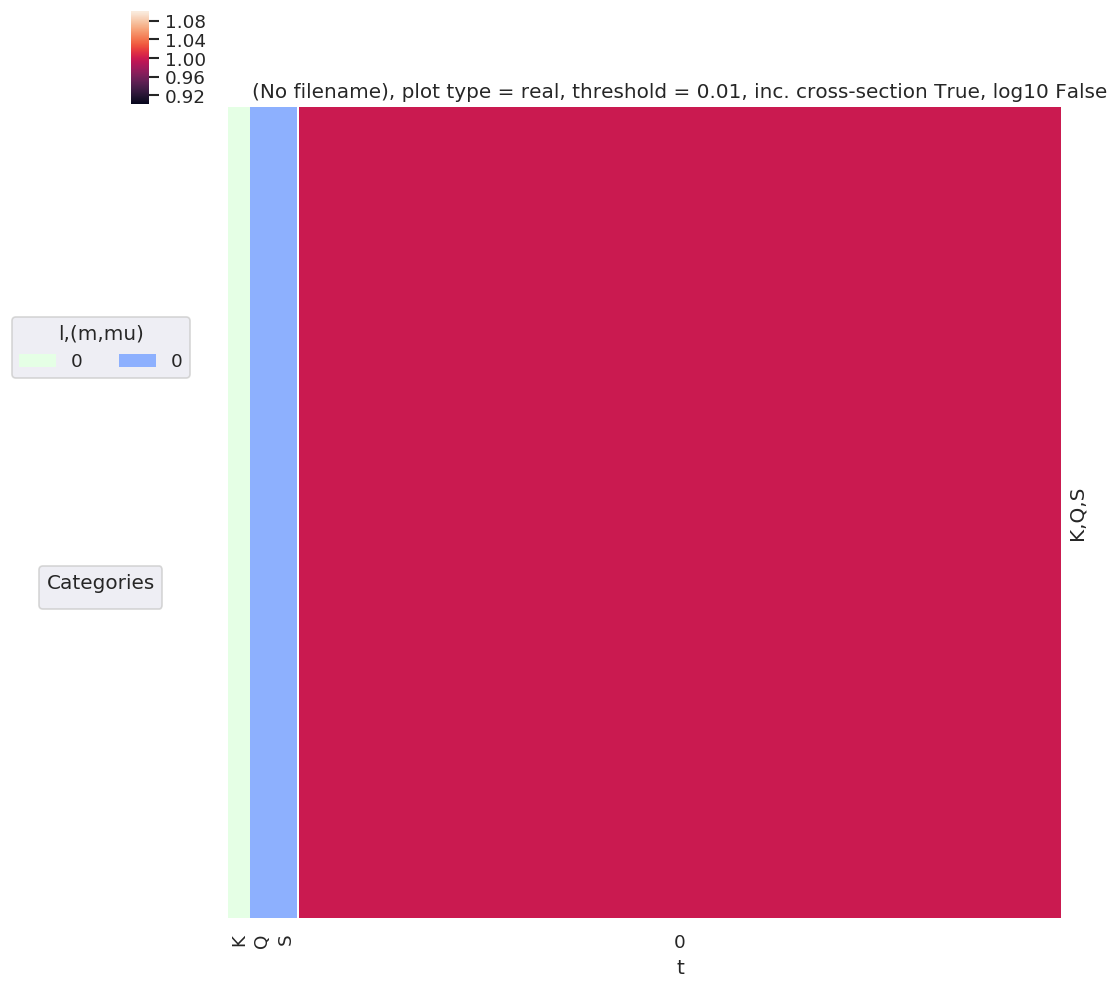:

# Test multiple t points
AKQS = ep.setADMs(ADMs = [0,0,0,1,1], t=[0,1])
daPlot, daPlotpd, legendList, gFig = ep.lmPlot(AKQS, xDim = 't', pType = 'r', squeeze = False)  # Note squeeze = False required for 1D case (should add this to code!)
daPlotpd

Plotting data (No filename), pType=r, thres=0.01, with Seaborn

No handles with labels found to put in legend.

:

t 0 1
K Q S
0 0 0 1.0 1.0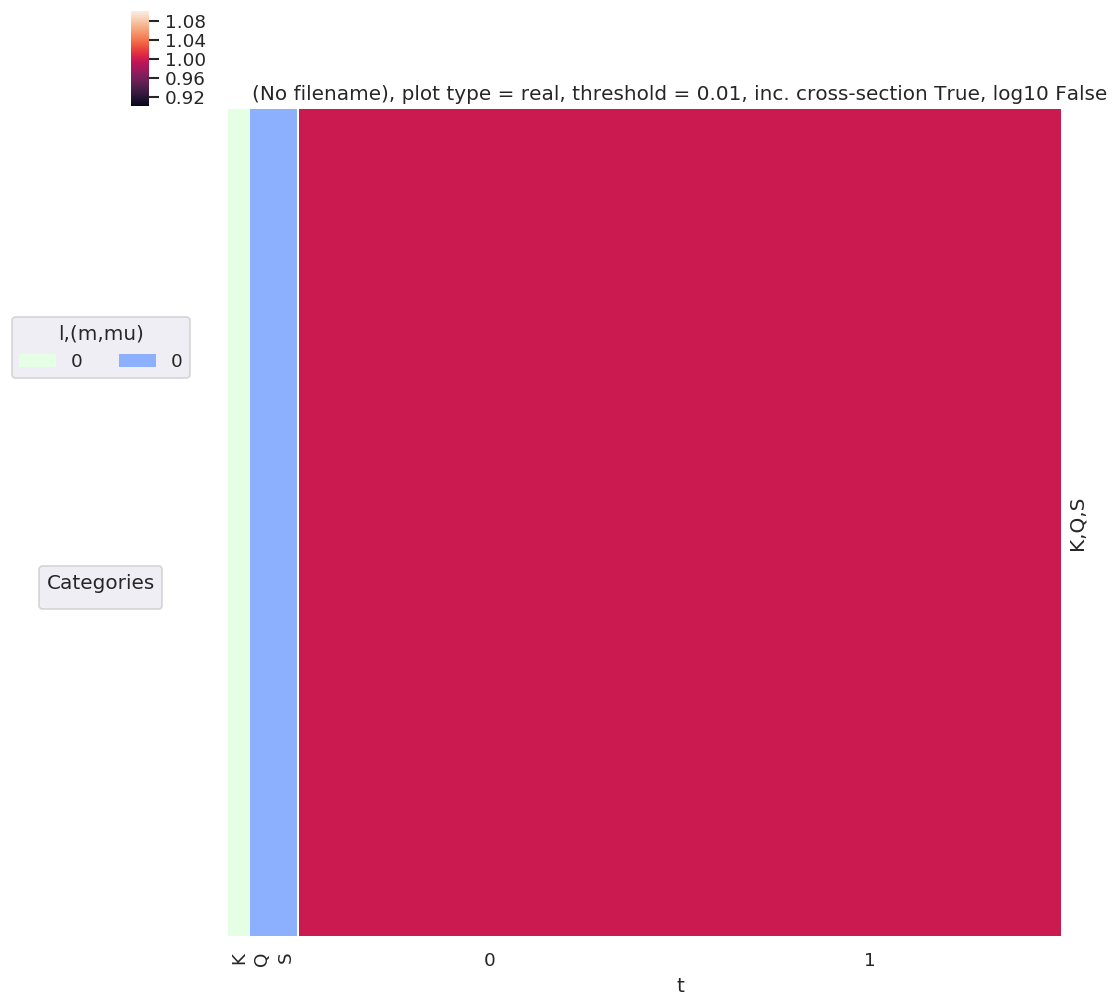:

# Test additional time-dependent values
AKQS = ep.setADMs(ADMs = [[0,0,0,1,1],[2,0,0,0,0.5]], t=[0,1])    # Nested list or np.array OK.
# AKQS = ep.setADMs(ADMs = np.array([[0,0,0,1,1],[2,0,0,0,0.5]]), t=[0,1])
daPlot, daPlotpd, legendList, gFig = ep.lmPlot(AKQS, xDim = 't', pType = 'r', squeeze = False)  # Note squeeze = False required for 1D case (should add this to code!)
daPlotpd

No handles with labels found to put in legend.

Plotting data (No filename), pType=r, thres=0.01, with Seaborn

:

t 0 1
K Q S
0 0 0 1.0 1.0
2 0 0 NaN 0.5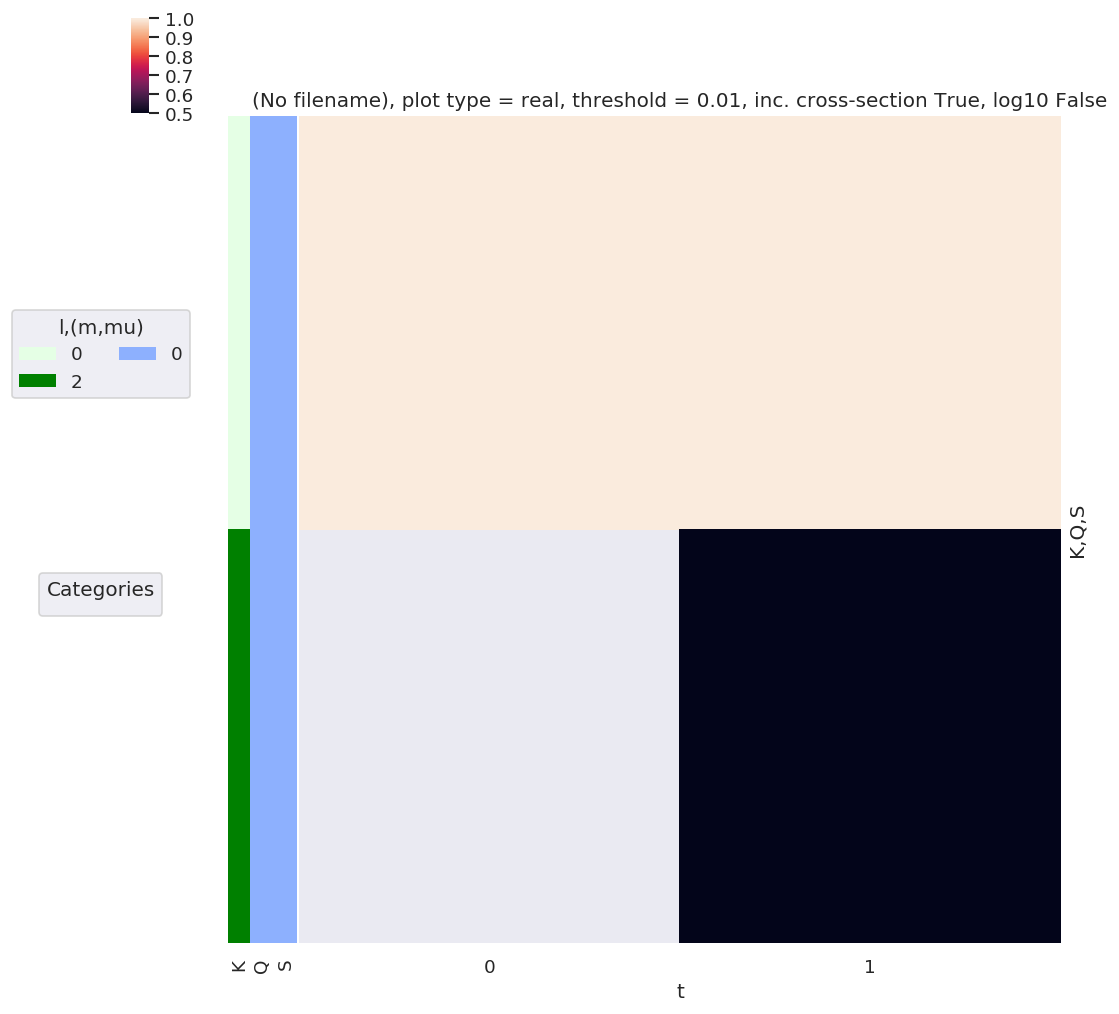### Alignment tensor¶

As previously defined:

\begin{equation} \Delta_{L,M}(K,Q,S)=(2K+1)^{1/2}(-1)^{K+Q}\left(\begin{array}{ccc} P & K & L\\ R & -Q & -M \end{array}\right)\left(\begin{array}{ccc} P & K & L\\ R' & -S & S-R' \end{array}\right) \end{equation}

#### Function dev¶

Based on existing MF functions, see ep.geomFunc.geomCalc and ep.geomFunc.mfblmGeom.

Most similar to existing betaTerm() function, which also has product of 3j terms in a similar manner.

:

# Generate QNs - code adapted from ep.geomFunc.geomUtils.genllL(Lmin = 0, Lmax = 10, mFlag = True):

# Generate QNs for deltaKQS term - 3j product term
def genKQSterms(Kmin = 0, Kmax = 2, mFlag = True):
# Set QNs for calculation, (l,m,mp)
QNs = []
for P in np.arange(0, 2+1):    # HARD-CODED R for testing - should get from EPR tensor defn. in full calcs.
for K in np.arange(Kmin, Kmax+1):  # Eventually this will come from alignment term
for L in np.arange(np.abs(P-K), P+K+1):  # Allowed L given P and K defined

if mFlag:    # Include "m" (or equivalent) terms?
mMax = L
RMax = P
QMax = K
else:
mMax = 0
RMax = 0
QMax = 0

for R in np.arange(-RMax, RMax+1):
for Q in np.arange(-QMax, QMax+1):
#for M in np.arange(np.abs(l-lp), l+lp+1):
#                         for M in np.arange(-mMax, mMax+1):
# Set M - note this implies specific phase choice.
# M = -(m+mp)
# M = (-m+mp)
# if np.abs(M) <= L:  # Skip terms with invalid M
#     QNs.append([l, lp, L, m, mp, M])

# Run for all possible M
for M in np.arange(-L, L+1):
QNs.append([P, K, L, R, Q, M])

return np.array(QNs)

# Generate QNs from EPR + AKQS tensors
def genKQStermsFromTensors(EPR, AKQS, uniqueFlag = True, phaseConvention = 'S'):
'''
Generate all QNs for :math:\Delta_{L,M}(K,Q,S) from existing tensors (Xarrays) :math:E_{P,R} and :math:A^K_{Q,S}.

Cf. :py:func:epsproc.geomFunc.genllpMatE, code adapted from there.

Parameters
----------
matE : Xarray
Xarray containing matrix elements, with QNs (l,m), as created by :py:func:readMatEle

uniqueFlag : bool, default = True
Check for duplicates and remove (can occur with some forms of matrix elements).

mFlag : bool, optional, default = True
m, mp take all passed values if mFlag=True, or =0 only if mFlag=False

phaseConvention : optional, str, default = 'S'
Set phase conventions with :py:func:epsproc.geomCalc.setPhaseConventions.
To use preset phase conventions, pass existing dictionary.
If matE.attrs['phaseCons'] is already set, this will be used instead of passed args.

Returns
-------
QNs1, QNs2 : two 2D np.arrays
Values take all allowed combinations ['P','K','L','R','Q','M'] and ['P','K','L','Rp','S','S-Rp'] from supplied matE.
Note phase conventions not applied to QN lists as yet.

To do
-----
- Implement output options (see dev. function w3jTable).

'''

# Local import.
from epsproc.geomFunc.geomCalc import setPhaseConventions

# For transparency/consistency with subfunctions, str/dict now set in setPhaseConventions()
if 'phaseCons' in EPR.attrs.keys():
phaseCons = EPR.attrs['phaseCons']
else:
phaseCons = setPhaseConventions(phaseConvention = phaseConvention)

# Get QNs from inputs
KQScoords = AKQS.unstack().coords  # Use unstack here, or np.unique(matE.l), to avoid duplicates
PRcoords = EPR.unstack().coords

# Use passed (m,mp) values, or run for m=mp=0 only.
#     if mFlag:
#         mList = matE.unstack().m.values
#     else:
#         mList = 0

# Set QNs for calculation, one set for each 3j term
QNs1 = []
QNs2 = []
for P in PRcoords['P'].values:   # Note dictionary syntax for coords objects
for K in KQScoords['K'].values:
for L in np.arange(np.abs(P-K), P+K+1):  # Allowed L given P and K defined

#                 if mFlag:    # Include "m" (or equivalent) terms?
#                     mMax = L
#                     RMax = P
#                     QMax = K
#                 else:
#                     mMax = 0
#                     RMax = 0
#                     QMax = 0

for R in PRcoords['R'].values:
for Q in KQScoords['Q'].values:

# Set M, with +/- phase convention - TBC MAY BE INCORRECT IN THIS CASE/CONTEXT?
# Note that setting phaseCons['afblmCons']['negM']  = phaseCons['genMatEcons']['negm'] is current default case, but doesn't have to be!
if phaseCons['genMatEcons']['negm']:
M = (-R+Q)  # Case for M -> -M switch
else:
M = -(R+Q)  # Usual phase convention.

QNs1.append([P, K, L, R, Q, M])

# Set Rp and S - these are essentially independent of R,Q,M, but keep nested for full dim output.
for Rp in PRcoords['R'].values:
for S in KQScoords['S'].values:
SRp = S-Rp  # Set final 3j term, S-Rp

QNs2.append([P, K, L, Rp, S, SRp])

#for M in np.arange(np.abs(l-lp), l+lp+1):
#                         for M in np.arange(-mMax, mMax+1):
# Set M - note this implies specific phase choice.
# M = -(m+mp)
# M = (-m+mp)
# if np.abs(M) <= L:  # Skip terms with invalid M
#     QNs.append([l, lp, L, m, mp, M])

# Run for all possible M
#                         for M in np.arange(-L, L+1):
#                             QNs.append([P, K, L, R, Q, M])

if uniqueFlag:
return np.unique(QNs1, axis = 0), np.unique(QNs2, axis = 0)
else:
return np.array(QNs1), np.array(QNs2)

:

test = genKQSterms()
# test.tolist()   # Use tolist() to get full array output (not truncated by np)
test.shape

:

(1225, 6)

:

# Can also just use existing fn.
test2 = ep.geomFunc.genllL(Lmax=2)
test2.shape

:

(1225, 6)

:

# Also QN list fn - checks and removes duplicates
QNs = ep.geomFunc.genllL(Lmax=2)
test3 = ep.geomFunc.geomUtils.genllLList(QNs, uniqueFlag = True, mFlag = True)

test3.shape

:

(1225, 6)


#### All QNs¶

:

# Then calc 3js.... as per betaTerm
form = 'xdaLM'  # xds
dlist1 = ['P', 'K', 'L', 'R', 'Q', 'M']
dlist2 = ['P', 'K', 'L', 'Rp', 'S', 'S-Rp']

QNs = ep.geomFunc.genllL(Lmax=2)

:

# Set phase conventions for this case, extending existing structure
phaseCons = ep.geomFunc.setPhaseConventions('E')

phaseCons['afblmCons'] = {}

# (+/-)M phase selection, set as per existing code, betaCons['negM'] = genMatEcons['negm']       # Use -M term in 3j? Should be anti-correlated with genMatEcons['negm']...? 31/03/20 NOW correlated with mfblmCons['Mphase']
# Note this is correlated with QN generation in genllpMatE() - should set equivalent fn for alignment terms.
# In existing case this arises from M = (-m+mp) or M = -(m+mp) choice.
phaseCons['afblmCons']['negM'] = phaseCons['genMatEcons']['negm']
phaseCons['afblmCons']['negQ'] = True
phaseCons['afblmCons']['negS'] = True

# Apply phase conventions to input QNs
#         if phaseCons['mfblmCons']['BLMmPhase']:
#             QNsBLMtable[:,3] *= -1
#             QNsBLMtable[:,5] *= -1

:

# Calculate two 3j terms, with respective QN sets
thrj1 = ep.geomFunc.w3jTable(QNs = QNs, nonzeroFlag = True, form = form, dlist = dlist1)
thrj2 = ep.geomFunc.w3jTable(QNs = QNs, nonzeroFlag = True, form = form, dlist = dlist2)

:

# Multiplication term...

testMult = thrj1.unstack() * thrj2.unstack()

# This can get large quickly - for Kmax=2 already have 2e6 terms

:

# plotDimsRed = ['l', 'm', 'lp', 'mp']
xDim = {'LM':['L','M']}
# daPlot, daPlotpd, legendList, gFig = ep.lmPlot(testMult, plotDims=plotDimsRed, xDim=xDim, pType = 'r')
daPlot, daPlotpd, legendList, gFig = ep.lmPlot(testMult, xDim=xDim, pType = 'r')

Set dataType (No dataType)
Plotting data (No filename), pType=r, thres=0.01, with Seaborn

C:\Users\femtolab\.conda\envs\ePSdev\lib\site-packages\xarray\core\nputils.py:215: RuntimeWarning:

All-NaN slice encountered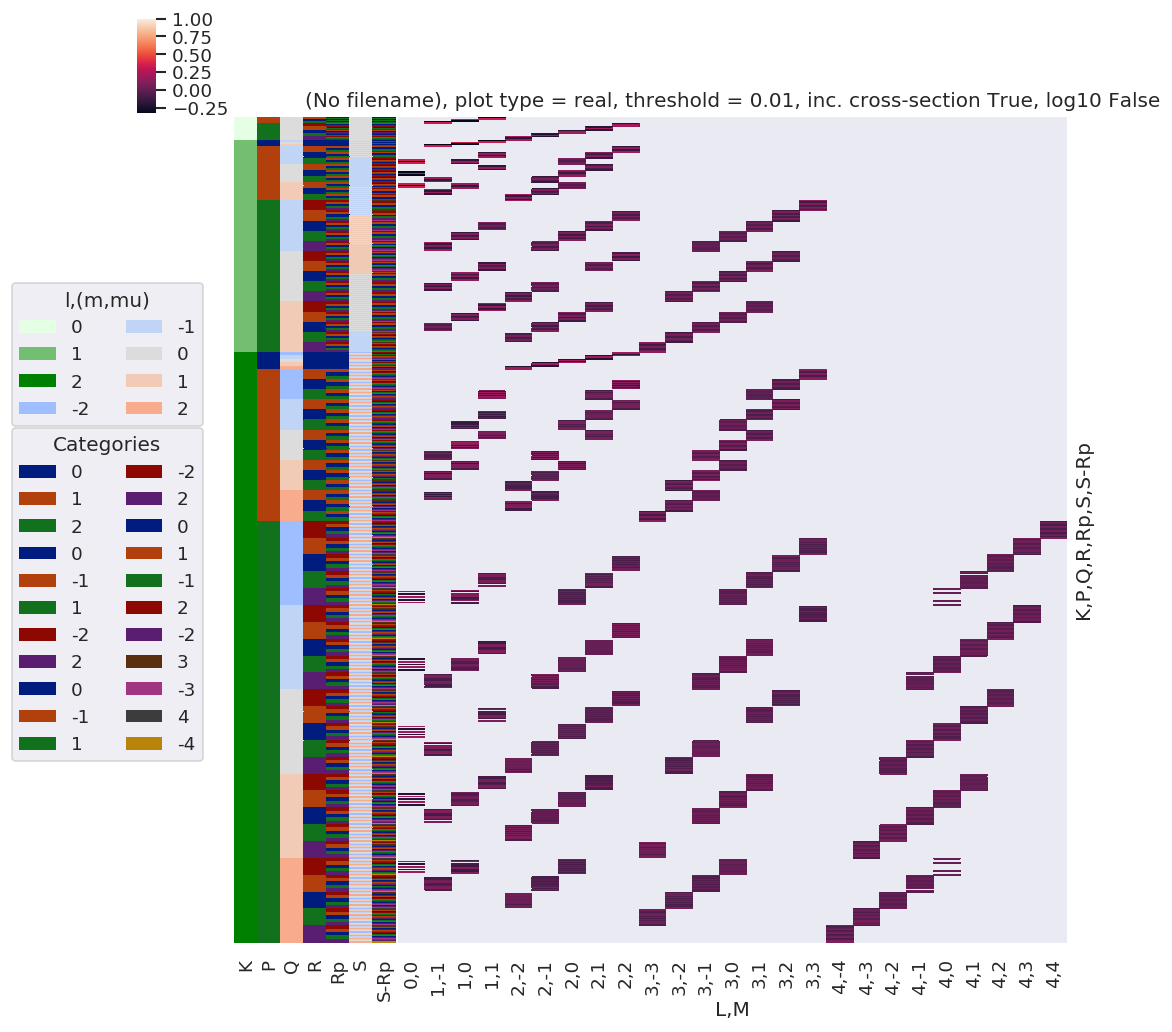#### Reduced QNs set¶

:

# Then calc 3js.... as per betaTerm
form = 'xdaLM'  # xds
dlist1 = ['P', 'K', 'L', 'R', 'Q', 'M']
dlist2 = ['P', 'K', 'L', 'Rp', 'S', 'S-Rp']

# Calculate two 3j terms, with respective QN sets
thrj1 = ep.geomFunc.w3jTable(QNs = QNs, nonzeroFlag = True, form = form, dlist = dlist1)
thrj2 = ep.geomFunc.w3jTable(QNs = QNs, nonzeroFlag = True, form = form, dlist = dlist2)

:

# With subselection (currently don't have QN generation fnc of correct form - see geomUtils)
# from epsproc.util import matEleSelector
# thrj1Sel = ep.util.matEleSelector(thrj1, inds = {'Q':0}, sq=False)

:

# Check 3j terms
xDim = {'LM':['L','M']}
daPlot, daPlotpd, legendList, gFig = ep.lmPlot(thrj1, xDim=xDim, pType = 'r')

xDim = {'LM':['L','S-Rp']}
daPlot, daPlotpd, legendList, gFig = ep.lmPlot(thrj2.sel({'S':0}), xDim=xDim, pType = 'r')

C:\Users\femtolab\.conda\envs\ePSdev\lib\site-packages\xarray\core\nputils.py:215: RuntimeWarning:

All-NaN slice encountered


Plotting data (No filename), pType=r, thres=0.01, with Seaborn

C:\Users\femtolab\.conda\envs\ePSdev\lib\site-packages\xarray\core\nputils.py:215: RuntimeWarning:

All-NaN slice encountered


Plotting data (No filename), pType=r, thres=0.01, with Seaborn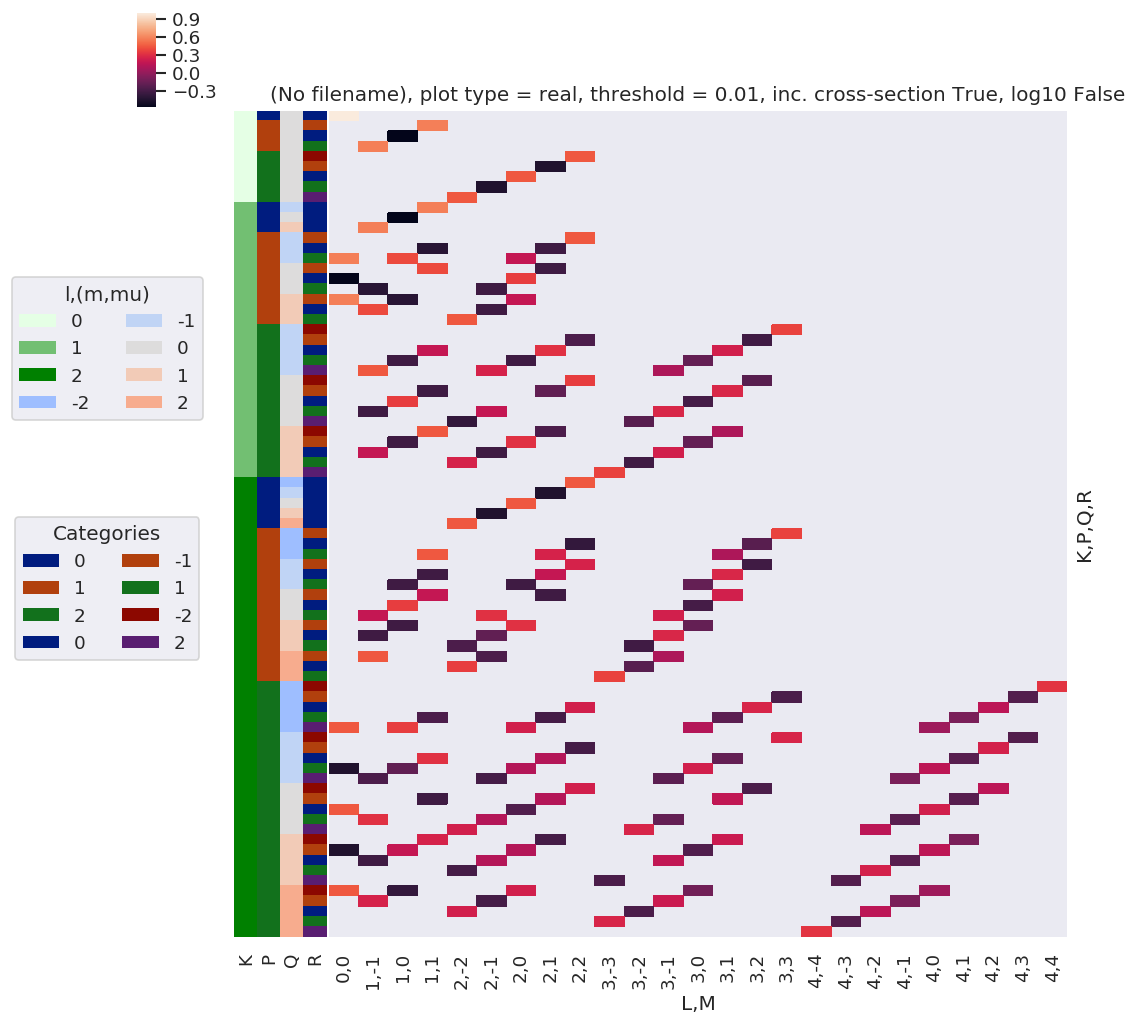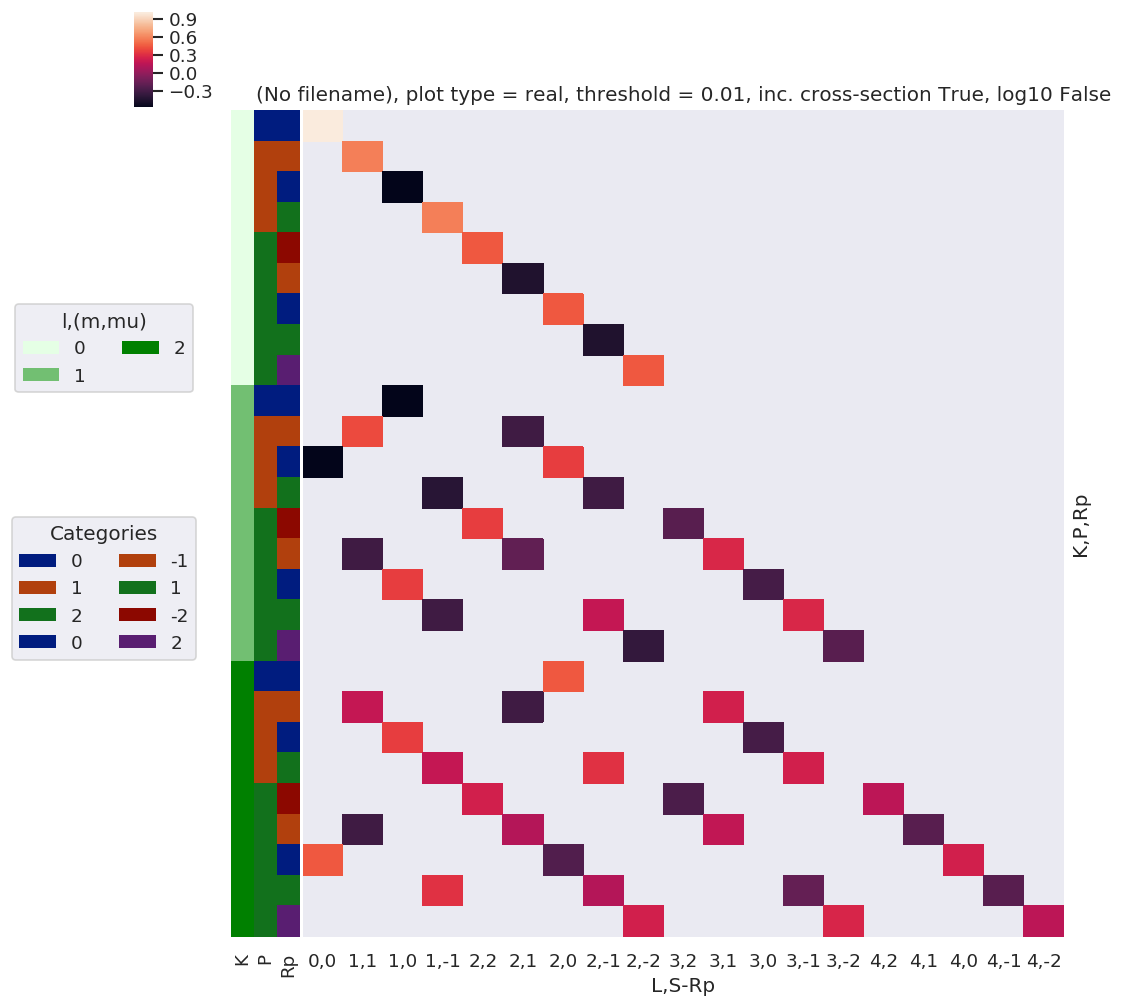:

# Multiplication term...

testMultSub = thrj1.unstack().sel({'Q':0}) * thrj2.unstack().sel({'S':0})   # SLOW, 91125 elements. ACTUALLY - much faster after a reboot. Sigh.

testMultSub2 = thrj1.sel({'Q':0}).unstack() * thrj2.sel({'S':0}).unstack()  # FAST, 28125 elements. Possible issue with which dims are dropped - check previous notes!

# This can get large quickly - for Kmax=2 already have 2e6 terms

testMultSub.notnull().sum() # == 194 for Kmax = 2, Q=S=0
testMultSub2.notnull().sum() # == 194 for Kmax = 2, Q=S=0  OK

:

<xarray.DataArray 'w3jStacked' ()>
array(194)
Coordinates:
Q        int64 0
S        int64 0
:

<xarray.DataArray 'w3jStacked' ()>
array(194)
:

# plotDimsRed = ['l', 'm', 'lp', 'mp']
xDim = {'LM':['L','M']}
# daPlot, daPlotpd, legendList, gFig = ep.lmPlot(testMult, plotDims=plotDimsRed, xDim=xDim, pType = 'r')
daPlot, daPlotpd, legendList, gFig = ep.lmPlot(testMultSub, xDim=xDim, pType = 'r')

Set dataType (No dataType)
Plotting data (No filename), pType=r, thres=0.01, with Seaborn

C:\Users\femtolab\.conda\envs\ePSdev\lib\site-packages\xarray\core\nputils.py:215: RuntimeWarning:

All-NaN slice encountered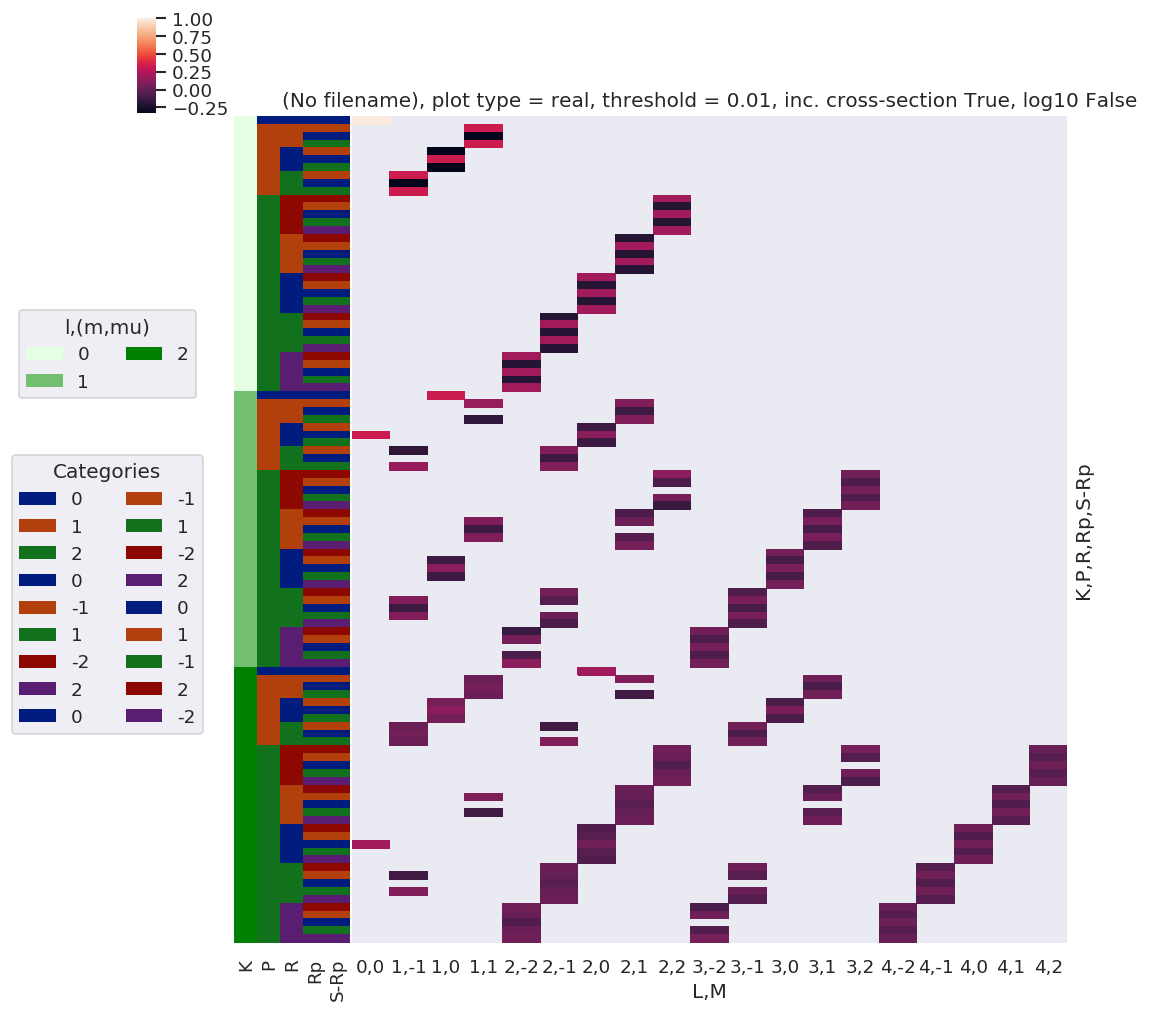:

# plotDimsRed = ['l', 'm', 'lp', 'mp']
xDim = {'LM':['L','M']}
# daPlot, daPlotpd, legendList, gFig = ep.lmPlot(testMult, plotDims=plotDimsRed, xDim=xDim, pType = 'r')
daPlot, daPlotpd, legendList, gFig = ep.lmPlot(testMultSub2, xDim=xDim, pType = 'r')

Set dataType (No dataType)
Plotting data (No filename), pType=r, thres=0.01, with Seaborn

C:\Users\femtolab\.conda\envs\ePSdev\lib\site-packages\xarray\core\nputils.py:215: RuntimeWarning:

All-NaN slice encountered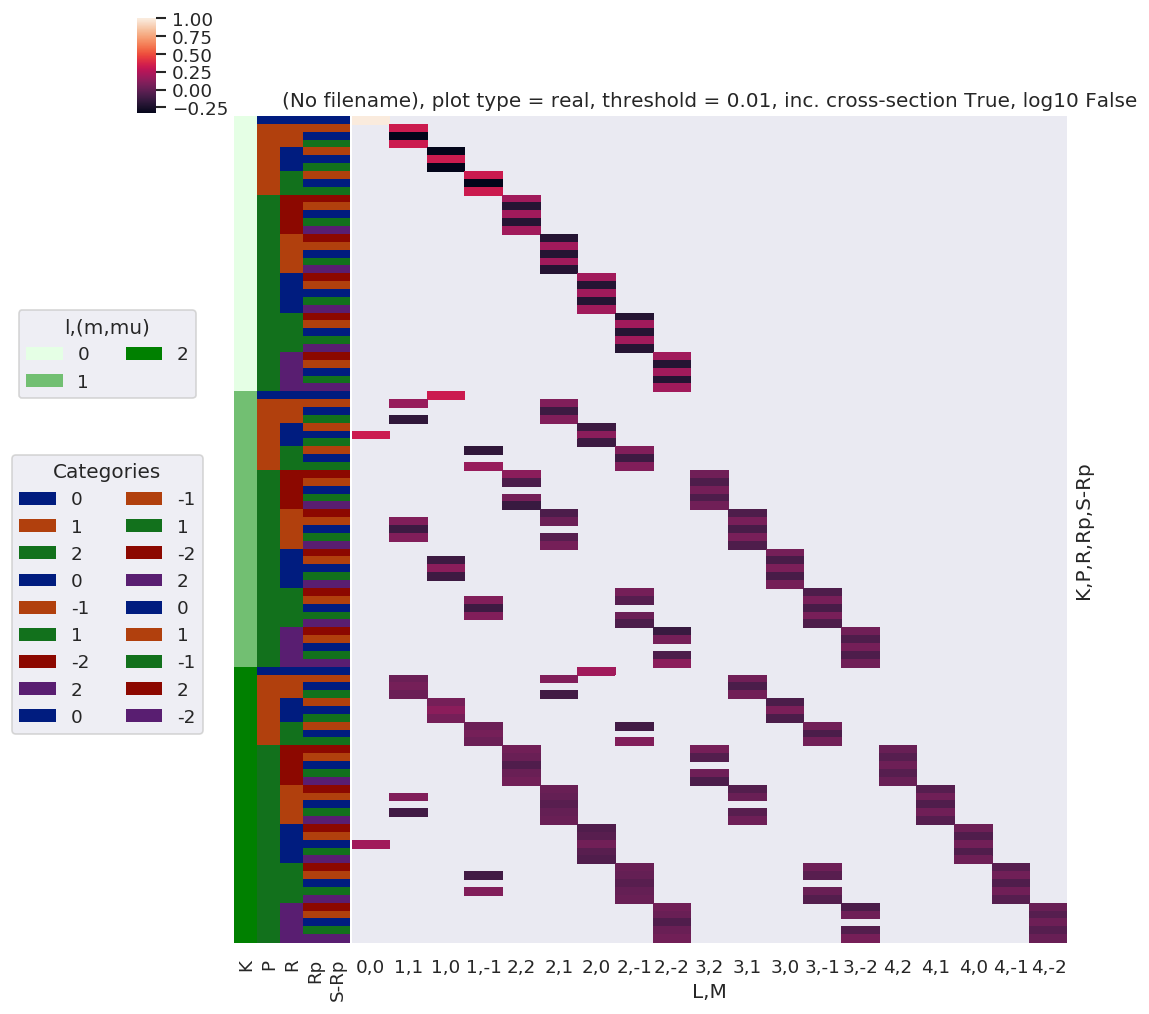Plots appear to be the same, aside from ordering of M terms(?)

### QNs from existing tensors¶

:

phaseConvention = 'E'

# Set polarisation term
p=
EPRX = geomCalc.EPR(form = 'xarray', p = p, nonzeroFlag = True, phaseConvention = phaseConvention).unstack().sel({'R-p':0}).drop('R-p') # Set for R-p = 0 for p=0 case (redundant coord) - need to fix in e-field mult term!
EPRXresort = EPRX.squeeze(['l','lp']).drop(['l','lp'])  # Safe squeeze & drop of selected singleton dims only.

# Set alignment terms (inc. time-dependence)
AKQS = ep.setADMs(ADMs = [[0,0,0,1,1],[2,0,0,0,0.5]], t=[0,1])    # Nested list or np.array OK.

:

# Calculate alignment term - this cell should form core function, cf. betaTerm() etc.

# Set QNs
QNs1, QNs2 = genKQStermsFromTensors(EPRXresort, AKQS, uniqueFlag = True, phaseConvention = phaseConvention)

# Then calc 3js.... as per betaTerm
form = 'xdaLM'  # xds
dlist1 = ['P', 'K', 'L', 'R', 'Q', 'M']
dlist2 = ['P', 'K', 'L', 'Rp', 'S', 'S-Rp']

# Copy QNs and apply any additional phase conventions
QNs1DeltaTable = QNs1.copy()
QNs2DeltaTable = QNs2.copy()

# Set additional phase cons here - these will be set in master function eventually!
# NOTE - only testing for Q=S=0 case initially.
phaseCons['afblmCons']['negM'] = phaseCons['genMatEcons']['negm']  # IF SET TO TRUE THIS KNOCKS OUT M!=0 terms - not sure if this is correct here, depends also on phase cons in genKQStermsFromTensors().
# Yeah, looks like phase error in current case, get terms with R=M, instead of R=-M
# Confusion is due to explicit assignment of +/-M terms in QN generation (only allowed terms), which *already* enforces this phase convention.
phaseCons['afblmCons']['negQ'] = True
phaseCons['afblmCons']['negS'] = True

# Switch signs (m,M) before 3j calcs.
if phaseCons['afblmCons']['negQ']:
QNs1DeltaTable[:,4] *= -1

# Switch sign Q > -Q before 3j calcs.
if phaseCons['afblmCons']['negM']:
QNs1DeltaTable[:,5] *= -1

# Switch sign S > -S before 3j calcs.
if phaseCons['afblmCons']['negS']:
QNs2DeltaTable[:,4] *= -1

# Calculate two 3j terms, with respective QN sets
thrj1 = ep.geomFunc.w3jTable(QNs = QNs1DeltaTable, nonzeroFlag = True, form = form, dlist = dlist1)
thrj2 = ep.geomFunc.w3jTable(QNs = QNs2DeltaTable, nonzeroFlag = True, form = form, dlist = dlist2)

# Multiply
thrjMult = thrj1.unstack() * thrj2.unstack()

# Additional terms & multiplications
Kdegen = np.sqrt(2*thrjMult.K + 1)
KQphase = np.power(-1, np.abs(thrjMult.K + thrjMult.Q))

DeltaKQS =  Kdegen * KQphase * thrjMult

# AF term
AFterm = (DeltaKQS * AKQS.unstack()).sum({'K','Q','S'})

:

thrjMult.notnull().sum() # == 69 for test case with all phase switches on, and same for no phase switches (in test case Q=S=0 in any case!)

:

<xarray.DataArray 'w3jStacked' ()>
array(69)
:

# Plot
xDim = {'LM':['L','M']}
# daPlot, daPlotpd, legendList, gFig = ep.lmPlot(testMult, plotDims=plotDimsRed, xDim=xDim, pType = 'r')
daPlot, daPlotpd, legendList, gFig = ep.lmPlot(DeltaKQS, xDim=xDim, pType = 'r')

Set dataType (No dataType)
Plotting data (No filename), pType=r, thres=0.01, with Seaborn

C:\Users\femtolab\.conda\envs\ePSdev\lib\site-packages\xarray\core\nputils.py:215: RuntimeWarning:

All-NaN slice encountered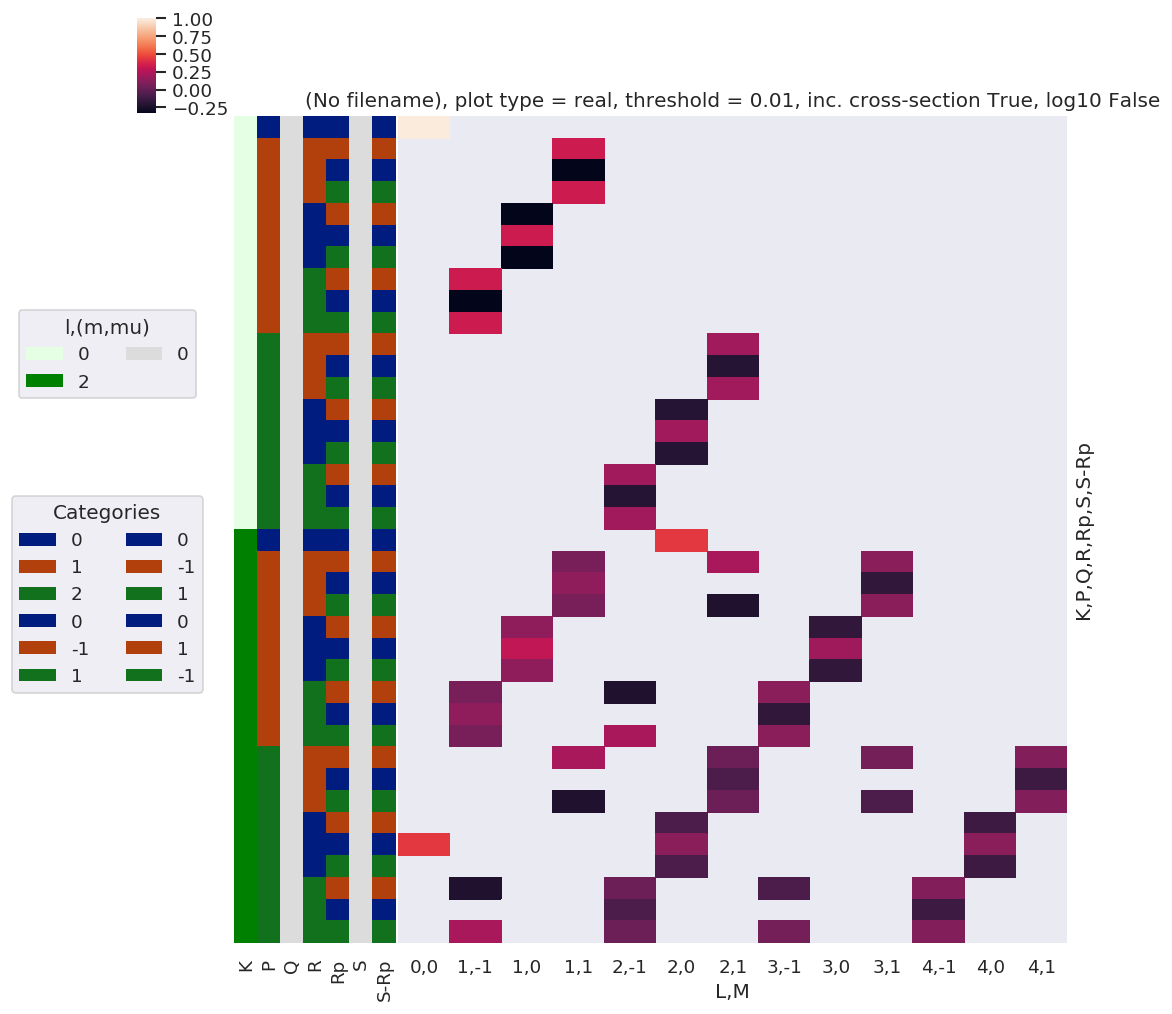:

# Plot
xDim = {'LM':['L','M']}
# daPlot, daPlotpd, legendList, gFig = ep.lmPlot(testMult, plotDims=plotDimsRed, xDim=xDim, pType = 'r')
daPlot, daPlotpd, legendList, gFig = ep.lmPlot(AFterm, xDim=xDim, pType = 'r')
daPlotpd

Set dataType (No dataType)
Plotting data (No filename), pType=r, thres=0.01, with Seaborn

No handles with labels found to put in legend.

:

L 0 1 2 3 4
M 0 -1 0 1 -1 0 1 -1 0 1 -1 0 1
P R Rp S-Rp t
0 0 0 0 0 1.000000 NaN NaN NaN NaN NaN NaN NaN NaN NaN NaN NaN NaN
1 1.000000 NaN NaN NaN NaN 0.223607 NaN NaN NaN NaN NaN NaN NaN
1 -1 -1 1 0 NaN NaN NaN 0.333333 NaN NaN NaN NaN NaN NaN NaN NaN NaN
1 NaN NaN NaN 0.370601 NaN NaN 0.111803 NaN NaN 0.063888 NaN NaN NaN
0 0 0 NaN NaN NaN -0.333333 NaN NaN NaN NaN NaN NaN NaN NaN NaN
1 NaN NaN NaN -0.258798 NaN NaN NaN NaN NaN -0.078246 NaN NaN NaN
1 -1 0 NaN NaN NaN 0.333333 NaN NaN NaN NaN NaN NaN NaN NaN NaN
1 NaN NaN NaN 0.370601 NaN NaN -0.111803 NaN NaN 0.063888 NaN NaN NaN
0 -1 1 0 NaN NaN -0.333333 NaN NaN NaN NaN NaN NaN NaN NaN NaN NaN
1 NaN NaN -0.258798 NaN NaN NaN NaN NaN -0.078246 NaN NaN NaN NaN
0 0 0 NaN NaN 0.333333 NaN NaN NaN NaN NaN NaN NaN NaN NaN NaN
1 NaN NaN 0.482405 NaN NaN NaN NaN NaN 0.095831 NaN NaN NaN NaN
1 -1 0 NaN NaN -0.333333 NaN NaN NaN NaN NaN NaN NaN NaN NaN NaN
1 NaN NaN -0.258798 NaN NaN NaN NaN NaN -0.078246 NaN NaN NaN NaN
1 -1 1 0 NaN 0.333333 NaN NaN NaN NaN NaN NaN NaN NaN NaN NaN NaN
1 NaN 0.370601 NaN NaN -0.111803 NaN NaN 0.063888 NaN NaN NaN NaN NaN
0 0 0 NaN -0.333333 NaN NaN NaN NaN NaN NaN NaN NaN NaN NaN NaN
1 NaN -0.258798 NaN NaN NaN NaN NaN -0.078246 NaN NaN NaN NaN NaN
1 -1 0 NaN 0.333333 NaN NaN NaN NaN NaN NaN NaN NaN NaN NaN NaN
1 NaN 0.370601 NaN NaN 0.111803 NaN NaN 0.063888 NaN NaN NaN NaN NaN
2 -1 -1 1 0 NaN NaN NaN NaN NaN NaN 0.200000 NaN NaN NaN NaN NaN NaN
1 NaN NaN NaN 0.111803 NaN NaN 0.215972 NaN NaN 0.031944 NaN NaN 0.053240
0 0 0 NaN NaN NaN NaN NaN NaN -0.200000 NaN NaN NaN NaN NaN NaN
1 NaN NaN NaN NaN NaN NaN -0.231944 NaN NaN NaN NaN NaN -0.058321
1 -1 0 NaN NaN NaN NaN NaN NaN 0.200000 NaN NaN NaN NaN NaN NaN
1 NaN NaN NaN -0.111803 NaN NaN 0.215972 NaN NaN -0.031944 NaN NaN 0.053240
0 -1 1 0 NaN NaN NaN NaN NaN -0.200000 NaN NaN NaN NaN NaN NaN NaN
1 NaN NaN NaN NaN NaN -0.231944 NaN NaN NaN NaN NaN -0.058321 NaN
0 0 0 NaN NaN NaN NaN NaN 0.200000 NaN NaN NaN NaN NaN NaN NaN
1 0.223607 NaN NaN NaN NaN 0.263888 NaN NaN NaN NaN NaN 0.063888 NaN
1 -1 0 NaN NaN NaN NaN NaN -0.200000 NaN NaN NaN NaN NaN NaN NaN
1 NaN NaN NaN NaN NaN -0.231944 NaN NaN NaN NaN NaN -0.058321 NaN
1 -1 1 0 NaN NaN NaN NaN 0.200000 NaN NaN NaN NaN NaN NaN NaN NaN
1 NaN -0.111803 NaN NaN 0.215972 NaN NaN -0.031944 NaN NaN 0.053240 NaN NaN
0 0 0 NaN NaN NaN NaN -0.200000 NaN NaN NaN NaN NaN NaN NaN NaN
1 NaN NaN NaN NaN -0.231944 NaN NaN NaN NaN NaN -0.058321 NaN NaN
1 -1 0 NaN NaN NaN NaN 0.200000 NaN NaN NaN NaN NaN NaN NaN NaN
1 NaN 0.111803 NaN NaN 0.215972 NaN NaN 0.031944 NaN NaN 0.053240 NaN NaN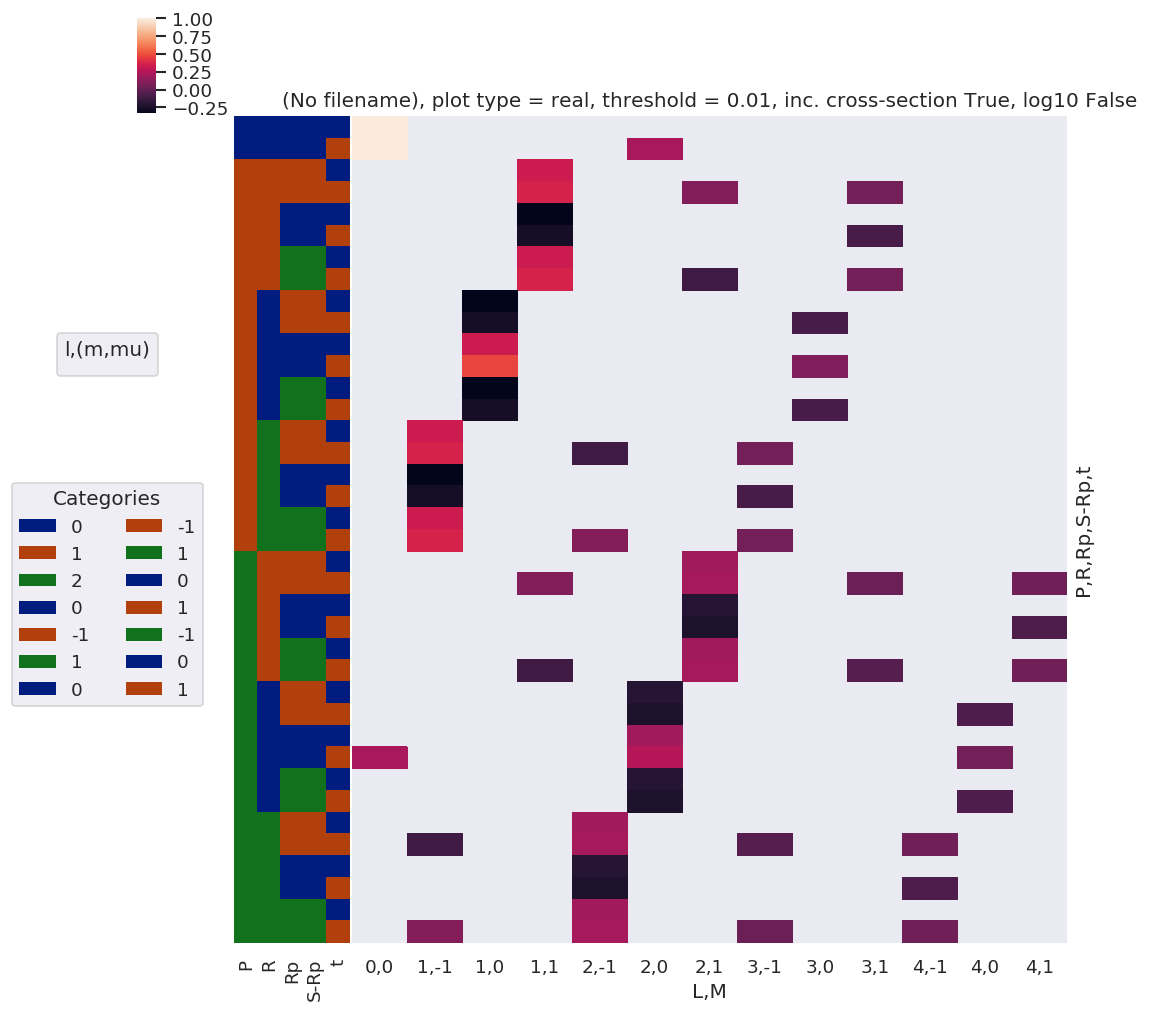Note here that there are blocks of non-zero terms with M=+/-1, these should drop out later (?) by sums over symmetry and/or other 3j terms… TBC…

## Lambda term redux¶

Use existing function and force/sub-select terms…

:

# Code adapted from mfblmXprod()

eulerAngs = np.array([0,0,0], ndmin=2)
# RX = ep.setPolGeoms(eulerAngs = eulerAngs)   # This throws error in geomCalc.MFproj???? Something to do with form of terms passed to wD, line 970 vs. 976 in geomCalc.py
RX = ep.setPolGeoms()   # (0,0,0) term in geomCalc.MFproj OK.

lambdaTerm, lambdaTable, lambdaD, QNsLambda = geomCalc.MFproj(RX = RX, form = 'xarray', phaseConvention = phaseConvention)
# lambdaTermResort = lambdaTerm.squeeze().drop('l').drop('lp')   # This removes photon (l,lp) dims fully.
lambdaTermResort = lambdaTerm.squeeze(['l','lp']).drop(['l','lp']).sel({'Labels':'z'})  # Safe squeeze & drop of selected singleton dims only.

:

# Plot
xDim = {'PRp':['P','Rp']}
# daPlot, daPlotpd, legendList, gFig = ep.lmPlot(testMult, plotDims=plotDimsRed, xDim=xDim, pType = 'r')
daPlot, daPlotpd, legendList, gFig = ep.lmPlot(lambdaTermResort, xDim=xDim, pType = 'r')
daPlotpd

Plotting data (No filename), pType=r, thres=0.01, with Seaborn

No handles with labels found to put in legend.

:

P 0 1 2
Rp 0 -1 0 1 -2 -1 0 1 2
R mu mup
-2 1 1 NaN NaN NaN NaN 0.447214 NaN NaN NaN NaN
-1 0 1 NaN -0.408248 NaN NaN NaN 0.316228 NaN NaN NaN
1 0 NaN 0.408248 NaN NaN NaN 0.316228 NaN NaN NaN
0 -1 1 0.57735 NaN -0.408248 NaN NaN NaN 0.182574 NaN NaN
0 0 -0.57735 NaN NaN NaN NaN NaN 0.365148 NaN NaN
1 -1 0.57735 NaN 0.408248 NaN NaN NaN 0.182574 NaN NaN
1 -1 0 NaN NaN NaN -0.408248 NaN NaN NaN 0.316228 NaN
0 -1 NaN NaN NaN 0.408248 NaN NaN NaN 0.316228 NaN
2 -1 -1 NaN NaN NaN NaN NaN NaN NaN NaN 0.447214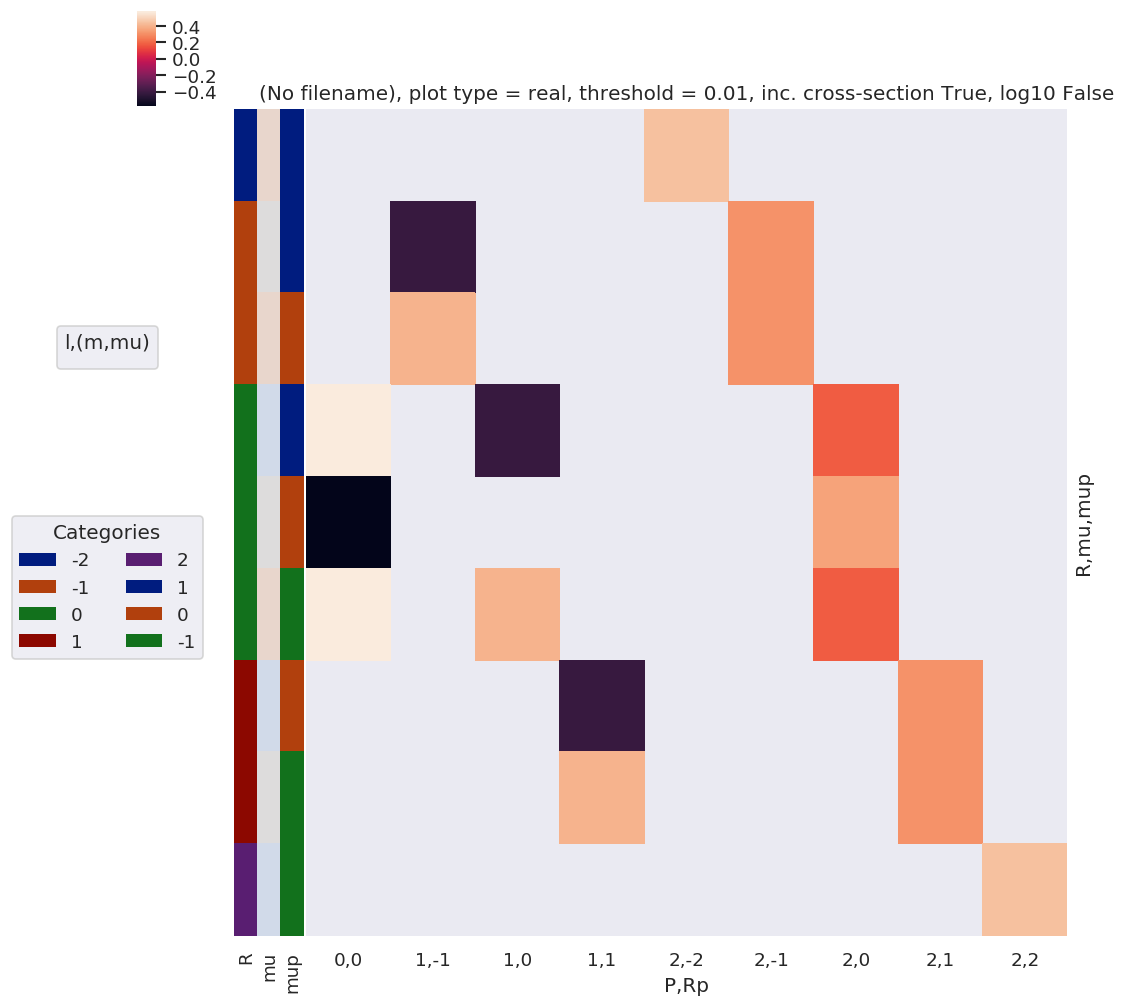### Check component terms are as expected¶

Should have R = Rp for z case, and wD terms = 1 or 0.

:

lambdaTablepd, _ = ep.util.multiDimXrToPD(lambdaTable, colDims=xDim, dropna=True)
lambdaTablepd

:

P 0 1 2
Rp 0 -1 0 1 -2 -1 0 1 2
R l lp mu mup
-2 1 1 -1 -1 NaN NaN NaN NaN NaN NaN NaN NaN 0.447214
0 NaN NaN NaN NaN NaN NaN NaN -0.316228 NaN
1 NaN NaN NaN NaN NaN NaN 0.182574 NaN NaN
0 -1 NaN NaN NaN NaN NaN NaN NaN -0.316228 NaN
0 NaN NaN NaN NaN NaN NaN 0.365148 NaN NaN
1 NaN NaN NaN NaN NaN -0.316228 NaN NaN NaN
1 -1 NaN NaN NaN NaN NaN NaN 0.182574 NaN NaN
0 NaN NaN NaN NaN NaN -0.316228 NaN NaN NaN
1 NaN NaN NaN NaN 0.447214 NaN NaN NaN NaN
-1 1 1 -1 -1 NaN NaN NaN NaN NaN NaN NaN NaN 0.447214
0 NaN NaN NaN 0.408248 NaN NaN NaN -0.316228 NaN
1 NaN NaN -0.408248 NaN NaN NaN 0.182574 NaN NaN
0 -1 NaN NaN NaN -0.408248 NaN NaN NaN -0.316228 NaN
0 NaN NaN NaN NaN NaN NaN 0.365148 NaN NaN
1 NaN 0.408248 NaN NaN NaN -0.316228 NaN NaN NaN
1 -1 NaN NaN 0.408248 NaN NaN NaN 0.182574 NaN NaN
0 NaN -0.408248 NaN NaN NaN -0.316228 NaN NaN NaN
1 NaN NaN NaN NaN 0.447214 NaN NaN NaN NaN
0 1 1 -1 -1 NaN NaN NaN NaN NaN NaN NaN NaN 0.447214
0 NaN NaN NaN 0.408248 NaN NaN NaN -0.316228 NaN
1 0.57735 NaN -0.408248 NaN NaN NaN 0.182574 NaN NaN
0 -1 NaN NaN NaN -0.408248 NaN NaN NaN -0.316228 NaN
0 -0.57735 NaN NaN NaN NaN NaN 0.365148 NaN NaN
1 NaN 0.408248 NaN NaN NaN -0.316228 NaN NaN NaN
1 -1 0.57735 NaN 0.408248 NaN NaN NaN 0.182574 NaN NaN
0 NaN -0.408248 NaN NaN NaN -0.316228 NaN NaN NaN
1 NaN NaN NaN NaN 0.447214 NaN NaN NaN NaN
1 1 1 -1 -1 NaN NaN NaN NaN NaN NaN NaN NaN 0.447214
0 NaN NaN NaN 0.408248 NaN NaN NaN -0.316228 NaN
1 NaN NaN -0.408248 NaN NaN NaN 0.182574 NaN NaN
0 -1 NaN NaN NaN -0.408248 NaN NaN NaN -0.316228 NaN
0 NaN NaN NaN NaN NaN NaN 0.365148 NaN NaN
1 NaN 0.408248 NaN NaN NaN -0.316228 NaN NaN NaN
1 -1 NaN NaN 0.408248 NaN NaN NaN 0.182574 NaN NaN
0 NaN -0.408248 NaN NaN NaN -0.316228 NaN NaN NaN
1 NaN NaN NaN NaN 0.447214 NaN NaN NaN NaN
2 1 1 -1 -1 NaN NaN NaN NaN NaN NaN NaN NaN 0.447214
0 NaN NaN NaN NaN NaN NaN NaN -0.316228 NaN
1 NaN NaN NaN NaN NaN NaN 0.182574 NaN NaN
0 -1 NaN NaN NaN NaN NaN NaN NaN -0.316228 NaN
0 NaN NaN NaN NaN NaN NaN 0.365148 NaN NaN
1 NaN NaN NaN NaN NaN -0.316228 NaN NaN NaN
1 -1 NaN NaN NaN NaN NaN NaN 0.182574 NaN NaN
0 NaN NaN NaN NaN NaN -0.316228 NaN NaN NaN
1 NaN NaN NaN NaN 0.447214 NaN NaN NaN NaN
:

lambdaTermResortpd, _ = ep.util.multiDimXrToPD(lambdaTermResort, colDims=xDim, dropna=True)
lambdaTermResortpd

# Think this is as per lambdaTable terms, just different ordering - because lambdaTable doesn't include some phase switches?

:

P 0 1 2
Rp 0 -1 0 1 -2 -1 0 1 2
R mu mup
-2 -1 -1 NaN NaN NaN NaN NaN NaN NaN NaN 0.000000+0.000000j
0 NaN NaN NaN NaN NaN NaN NaN 0.000000+0.000000j NaN
1 NaN NaN NaN NaN NaN NaN 0.000000+0.000000j NaN NaN
0 -1 NaN NaN NaN NaN NaN NaN NaN 0.000000+0.000000j NaN
0 NaN NaN NaN NaN NaN NaN 0.000000+0.000000j NaN NaN
1 NaN NaN NaN NaN NaN 0.000000+0.000000j NaN NaN NaN
1 -1 NaN NaN NaN NaN NaN NaN 0.000000+0.000000j NaN NaN
0 NaN NaN NaN NaN NaN 0.000000+0.000000j NaN NaN NaN
1 NaN NaN NaN NaN 0.447214+0.000000j NaN NaN NaN NaN
-1 -1 -1 NaN NaN NaN NaN NaN NaN NaN NaN 0.000000+0.000000j
0 NaN NaN NaN 0.000000+0.000000j NaN NaN NaN 0.000000+0.000000j NaN
1 NaN NaN 0.000000+0.000000j NaN NaN NaN 0.000000+0.000000j NaN NaN
0 -1 NaN NaN NaN 0.000000+0.000000j NaN NaN NaN 0.000000+0.000000j NaN
0 NaN NaN NaN NaN NaN NaN 0.000000+0.000000j NaN NaN
1 NaN -0.408248+0.000000j NaN NaN NaN 0.316228+0.000000j NaN NaN NaN
1 -1 NaN NaN 0.000000+0.000000j NaN NaN NaN 0.000000+0.000000j NaN NaN
0 NaN 0.408248+0.000000j NaN NaN NaN 0.316228+0.000000j NaN NaN NaN
1 NaN NaN NaN NaN 0.000000+0.000000j NaN NaN NaN NaN
0 -1 -1 NaN NaN NaN NaN NaN NaN NaN NaN 0.000000+0.000000j
0 NaN NaN NaN 0.000000+0.000000j NaN NaN NaN 0.000000+0.000000j NaN
1 0.577350+0.000000j NaN -0.408248+0.000000j NaN NaN NaN 0.182574+0.000000j NaN NaN
0 -1 NaN NaN NaN 0.000000+0.000000j NaN NaN NaN 0.000000+0.000000j NaN
0 -0.577350+0.000000j NaN NaN NaN NaN NaN 0.365148+0.000000j NaN NaN
1 NaN 0.000000+0.000000j NaN NaN NaN 0.000000+0.000000j NaN NaN NaN
1 -1 0.577350+0.000000j NaN 0.408248+0.000000j NaN NaN NaN 0.182574+0.000000j NaN NaN
0 NaN 0.000000+0.000000j NaN NaN NaN 0.000000+0.000000j NaN NaN NaN
1 NaN NaN NaN NaN 0.000000+0.000000j NaN NaN NaN NaN
1 -1 -1 NaN NaN NaN NaN NaN NaN NaN NaN 0.000000+0.000000j
0 NaN NaN NaN -0.408248+0.000000j NaN NaN NaN 0.316228+0.000000j NaN
1 NaN NaN 0.000000+0.000000j NaN NaN NaN 0.000000+0.000000j NaN NaN
0 -1 NaN NaN NaN 0.408248+0.000000j NaN NaN NaN 0.316228+0.000000j NaN
0 NaN NaN NaN NaN NaN NaN 0.000000+0.000000j NaN NaN
1 NaN 0.000000+0.000000j NaN NaN NaN 0.000000+0.000000j NaN NaN NaN
1 -1 NaN NaN 0.000000+0.000000j NaN NaN NaN 0.000000+0.000000j NaN NaN
0 NaN 0.000000+0.000000j NaN NaN NaN 0.000000+0.000000j NaN NaN NaN
1 NaN NaN NaN NaN 0.000000+0.000000j NaN NaN NaN NaN
2 -1 -1 NaN NaN NaN NaN NaN NaN NaN NaN 0.447214+0.000000j
0 NaN NaN NaN NaN NaN NaN NaN 0.000000+0.000000j NaN
1 NaN NaN NaN NaN NaN NaN 0.000000+0.000000j NaN NaN
0 -1 NaN NaN NaN NaN NaN NaN NaN 0.000000+0.000000j NaN
0 NaN NaN NaN NaN NaN NaN 0.000000+0.000000j NaN NaN
1 NaN NaN NaN NaN NaN 0.000000+0.000000j NaN NaN NaN
1 -1 NaN NaN NaN NaN NaN NaN 0.000000+0.000000j NaN NaN
0 NaN NaN NaN NaN NaN 0.000000+0.000000j NaN NaN NaN
1 NaN NaN NaN NaN 0.000000+0.000000j NaN NaN NaN NaN
:

# wigner D term looks good.
lambdaDpd, _ = ep.util.multiDimXrToPD(lambdaD.sel({'Labels':'z'}), colDims=xDim, dropna=True)
lambdaDpd


:

P 0 1 2
Rp 2 1 0 -1 -2 2 1 0 -1 -2 2 1 0 -1 -2
R
2 NaN NaN NaN NaN NaN NaN NaN NaN NaN NaN 1.000000-0.000000j 0.000000-0.000000j 0.000000-0.000000j 0.000000-0.000000j 0.000000-0.000000j
1 NaN NaN NaN NaN NaN 0.000000-0.000000j 1.000000-0.000000j 0.000000-0.000000j 0.000000-0.000000j 0.000000-0.000000j 0.000000-0.000000j 1.000000-0.000000j 0.000000-0.000000j 0.000000-0.000000j 0.000000-0.000000j
0 0.000000-0.000000j 0.000000-0.000000j 1.000000-0.000000j 0.000000-0.000000j 0.000000-0.000000j 0.000000-0.000000j 0.000000-0.000000j 1.000000-0.000000j 0.000000-0.000000j 0.000000-0.000000j 0.000000-0.000000j 0.000000-0.000000j 1.000000-0.000000j 0.000000-0.000000j 0.000000-0.000000j
-1 NaN NaN NaN NaN NaN 0.000000-0.000000j 0.000000-0.000000j 0.000000-0.000000j 1.000000+0.000000j 0.000000-0.000000j 0.000000-0.000000j 0.000000-0.000000j 0.000000-0.000000j 1.000000+0.000000j 0.000000-0.000000j
-2 NaN NaN NaN NaN NaN NaN NaN NaN NaN NaN 0.000000-0.000000j 0.000000-0.000000j 0.000000-0.000000j 0.000000-0.000000j 1.000000+0.000000j

## Build full calculation from functions¶

Use mfblmGeom.py as template: basically just need modified lambda term as above, and new alignment term, and rest of calculation should be identical.

:

# Check polProd term - incorporate alignment term here...?

# Existing terms
# polProd = (EPRXresort * lambdaTermResort)

# sumDimsPol = ['P','R','Rp','p']
# polProd = polProd.sum(sumDimsPol)

# Test with alignment term
polProd = (EPRXresort * lambdaTermResort * AFterm)
sumDimsPol = ['P','R','Rp','p', 'S-Rp']
polProd = polProd.sum(sumDimsPol)
# Looks OK - keeps correct dims in test case! 270 terms.


NOW implemented in ep.geomFunc.afblmGeom.py

## Testing…¶

16/06/20

Test code adapted from previous round of AF tests, http://localhost:8888/lab/tree/dev/ePSproc/ePSproc_AFBLM_calcs_bench_100220.ipynb

### Load data¶

:

# Load data from modPath\data
dataPath = os.path.join(modPath, 'data', 'photoionization')
dataFile = os.path.join(dataPath, 'n2_3sg_0.1-50.1eV_A2.inp.out')  # Set for sample N2 data for testing

# Scan data file
dataSet = ep.readMatEle(fileIn = dataFile)
dataXS = ep.readMatEle(fileIn = dataFile, recordType = 'CrossSection')  # XS info currently not set in NO2 sample file.

*** ePSproc readMatEle(): scanning files for DumpIdy segments.

*** Scanning file(s)
['D:\\code\\github\\ePSproc\\data\\photoionization\\n2_3sg_0.1-50.1eV_A2.inp.out']

*** Reading ePS output file:  D:\code\github\ePSproc\data\photoionization\n2_3sg_0.1-50.1eV_A2.inp.out
Expecting 51 energy points.
Expecting 2 symmetries.
Scanning CrossSection segments.
Expecting 102 DumpIdy segments.
Found 102 dumpIdy segments (sets of matrix elements).

Processing segments to Xarrays...
Processed 102 sets of DumpIdy file segments, (0 blank)
*** ePSproc readMatEle(): scanning files for CrossSection segments.

*** Scanning file(s)
['D:\\code\\github\\ePSproc\\data\\photoionization\\n2_3sg_0.1-50.1eV_A2.inp.out']

*** Reading ePS output file:  D:\code\github\ePSproc\data\photoionization\n2_3sg_0.1-50.1eV_A2.inp.out
Expecting 51 energy points.
Expecting 2 symmetries.
Scanning CrossSection segments.
Expecting 3 CrossSection segments.
Found 3 CrossSection segments (sets of results).
Processed 3 sets of CrossSection file segments, (0 blank)


### Plot ePS results (isotropic case)¶

:

# Plot cross sections using Xarray functionality
# Set here to plot per file - should add some logic to combine files.
for data in dataXS:
daPlot = data.sel(XC='SIGMA')
daPlot.plot.line(x='Eke', col='Type')

:

<xarray.plot.facetgrid.FacetGrid at 0x2c402402630>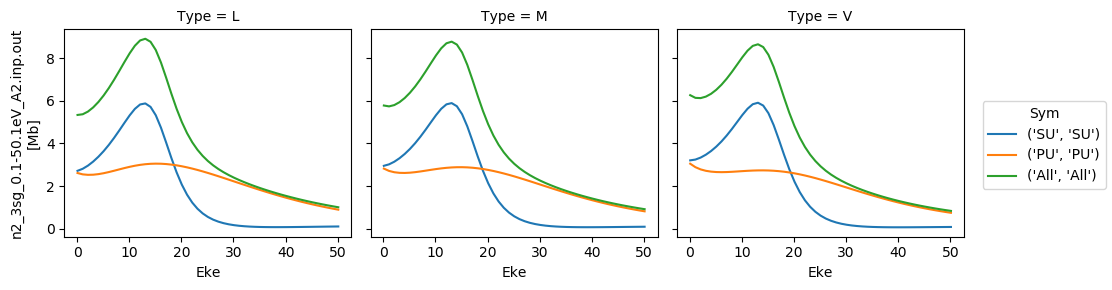:

# Repeat for betas
for data in dataXS:
daPlot = data.sel(XC='BETA')
daPlot.plot.line(x='Eke', col='Type')

:

<xarray.plot.facetgrid.FacetGrid at 0x2c402584828>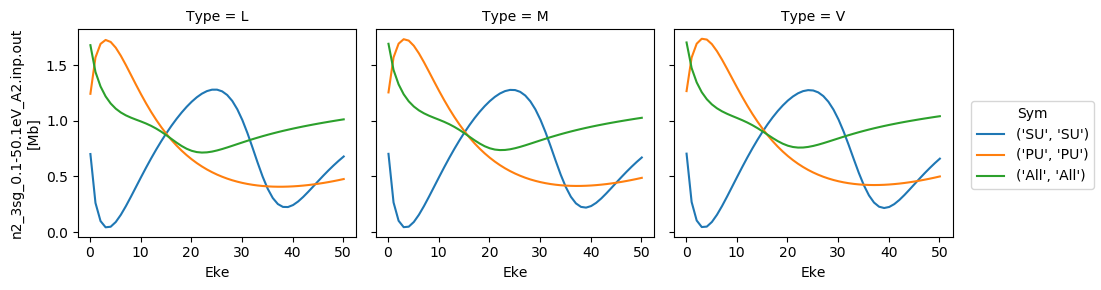### Try new AF calculation - isotropic (default) case¶

:

# Tabulate & plot matrix elements vs. Eke
selDims = {'it':1, 'Type':'L'}
matE = dataSet.sel(selDims)  # Set for N2 case, length-gauge results only.
daPlot, daPlotpd, legendList, gFig = ep.lmPlot(matE, xDim = 'Eke', pType = 'r', fillna = True)
daPlotpd

Plotting data n2_3sg_0.1-50.1eV_A2.inp.out, pType=r, thres=0.01, with Seaborn

:

Eke 0.1 1.1 2.1 3.1 4.1 5.1 6.1 7.1 8.1 9.1 ... 41.1 42.1 43.1 44.1 45.1 46.1 47.1 48.1 49.1 50.1
Cont Targ Total l m mu
PU SG PU 1 -1 1 -6.203556 7.496908 3.926892 1.071093 -0.335132 -1.043391 -1.396885 -1.554984 -1.598812 -1.573327 ... -0.396306 -0.422225 -0.448003 -0.473466 -0.498463 -0.522867 -0.546573 -0.569493 -0.591558 -0.612712
1 -1 -6.203556 7.496908 3.926892 1.071093 -0.335132 -1.043391 -1.396885 -1.554984 -1.598812 -1.573327 ... -0.396306 -0.422225 -0.448003 -0.473466 -0.498463 -0.522867 -0.546573 -0.569493 -0.591558 -0.612712
3 -1 1 -2.090641 -1.723467 -3.571018 -1.703191 0.232612 1.862713 3.201022 4.298839 5.198215 5.929303 ... 1.200463 1.040074 0.892501 0.757189 0.633556 0.521004 0.418928 0.326726 0.243800 0.169566
1 -1 -2.090641 -1.723467 -3.571018 -1.703191 0.232612 1.862713 3.201022 4.298839 5.198215 5.929303 ... 1.200463 1.040074 0.892501 0.757189 0.633556 0.521004 0.418928 0.326726 0.243800 0.169566
5 -1 1 0.000000 0.013246 0.000000 -0.013096 -0.024367 -0.033455 -0.042762 -0.053765 -0.067354 -0.083946 ... -0.325544 -0.318791 -0.312332 -0.306211 -0.300456 -0.295091 -0.290130 -0.285584 -0.281453 -0.277737
1 -1 0.000000 0.013246 0.000000 -0.013096 -0.024367 -0.033455 -0.042762 -0.053765 -0.067354 -0.083946 ... -0.325544 -0.318791 -0.312332 -0.306211 -0.300456 -0.295091 -0.290130 -0.285584 -0.281453 -0.277737
7 -1 1 0.000000 0.000000 0.000000 0.000000 0.000000 0.000000 0.000000 0.000000 0.000000 0.000000 ... 0.027469 0.028083 0.028678 0.029257 0.029823 0.030377 0.030923 0.031461 0.031994 0.032523
1 -1 0.000000 0.000000 0.000000 0.000000 0.000000 0.000000 0.000000 0.000000 0.000000 0.000000 ... 0.027469 0.028083 0.028678 0.029257 0.029823 0.030377 0.030923 0.031461 0.031994 0.032523
SU SG SU 1 0 0 6.246520 -10.081763 -8.912214 -5.342049 -3.160539 -1.795783 -0.867993 -0.174154 0.400643 0.926420 ... -0.854886 -0.940017 -1.021577 -1.099776 -1.174787 -1.246759 -1.315814 -1.382058 -1.445582 -1.506467
3 0 0 2.605768 2.775108 4.733473 1.677812 -1.450830 -4.187395 -6.554491 -8.601759 -10.343109 -11.747922 ... -0.293053 -0.441416 -0.579578 -0.708075 -0.827415 -0.938075 -1.040506 -1.135134 -1.222361 -1.302566
5 0 0 0.000000 -0.020864 0.000000 0.024263 0.042694 0.059142 0.077308 0.099330 0.126227 0.157745 ... 0.490499 0.511546 0.531810 0.551281 0.569951 0.587815 0.604868 0.621107 0.636528 0.651132
7 0 0 0.000000 0.000000 0.000000 0.000000 0.000000 0.000000 0.000000 0.000000 0.000000 0.000000 ... -0.039566 -0.041422 -0.043265 -0.045092 -0.046901 -0.048689 -0.050453 -0.052190 -0.053899 -0.055577

12 rows × 51 columns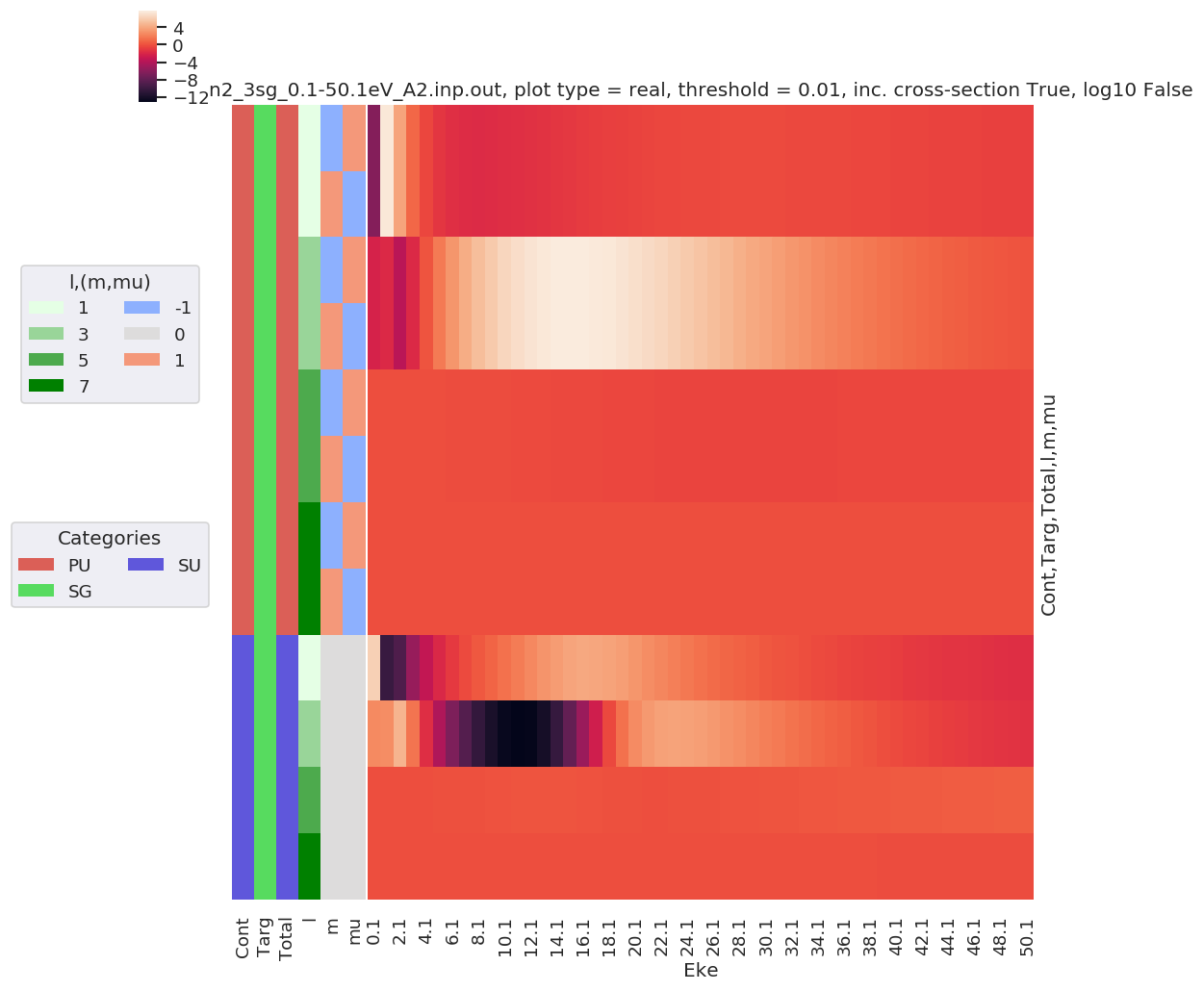:

phaseConvention = 'E'  # Set phase conventions used in the numerics - for ePolyScat matrix elements, set to 'E', to match defns. above.

symSum = False  # Sum over symmetry groups, or keep separate?
SFflag = True  # Include scaling factor to Mb in calculation?
thres = 1e-4
RX = ep.setPolGeoms()  # Set default pol geoms (z,x,y), or will be set by mfblmXprod() defaults - FOR AF case this is only used to set 'z' geom for unity wigner D's - should rationalise this!

start = time.time()
mTermST, mTermS, mTermTest = ep.geomFunc.afblmXprod(dataSet, QNs = None, RX=RX, thres = thres, selDims = {'it':1, 'Type':'L'}, thresDims='Eke', symSum=symSum, SFflag=True, phaseConvention=phaseConvention)
end = time.time()
print('Elapsed time = {0} seconds, for {1} energy points, {2} polarizations, threshold={3}.'.format((end-start), mTermST.Eke.size, RX.size, thres))

# Elapsed time = 3.3885273933410645 seconds, for 51 energy points, 3 polarizations, threshold=0.01.
# Elapsed time = 5.059587478637695 seconds, for 51 energy points, 3 polarizations, threshold=0.0001.

Elapsed time = 2.529463768005371 seconds, for 51 energy points, 3 polarizations, threshold=0.0001.

:

# Full results (before summation)
mTermST.attrs['dataType'] = 'matE'  # Set matE here to allow for correct plotting of sym dims.

plotDimsRed = ['Labels','L','M']  # Set plotDims to fix dim ordering in plot
if not symSum:
plotDimsRed.extend(['Cont','Targ','Total'])

daPlot, daPlotpd, legendList, gFig = ep.lmPlot(mTermST, plotDims=plotDimsRed, xDim='Eke', sumDims=None, pType = 'r', thres = 0.01, fillna = True, SFflag=False)

# daPlot, daPlotpd, legendList, gFig = ep.lmPlot(mTermST, xDim='Eke', sumDims=None, pType = 'r', thres = 0.01, fillna = True)  # If plotDims is not passed use default ordering.

Plotting data n2_3sg_0.1-50.1eV_A2.inp.out, pType=r, thres=0.01, with Seaborn

No handles with labels found to put in legend.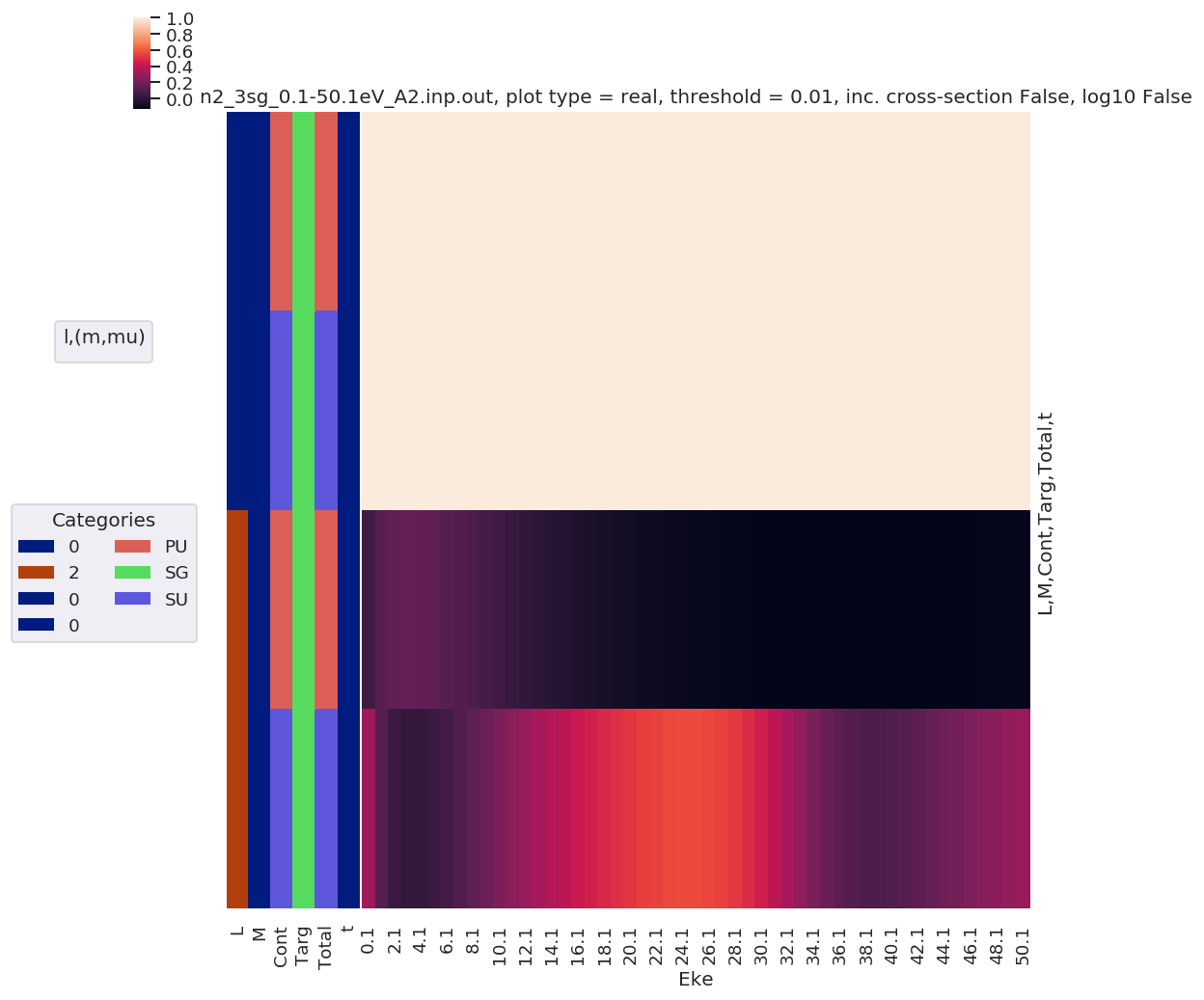:

mTermST.XS.real.squeeze().plot.line(x='Eke', col='Sym');
ep.util.matEleSelector(mTermST, thres = 0.1, dims='Eke').real.squeeze().plot.line(x='Eke', col='Sym');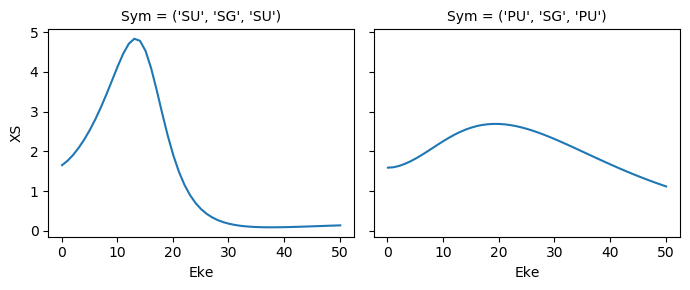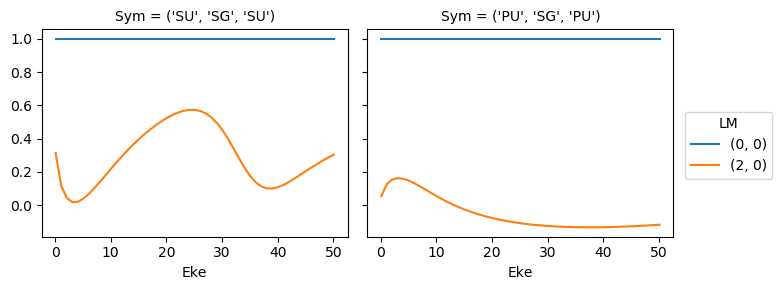### Try sym summation…¶

:

phaseConvention = 'E'  # Set phase conventions used in the numerics - for ePolyScat matrix elements, set to 'E', to match defns. above.

symSum = True  # Sum over symmetry groups, or keep separate?
SFflag = True  # Include scaling factor to Mb in calculation?
thres = 1e-4
RX = ep.setPolGeoms()  # Set default pol geoms (z,x,y), or will be set by mfblmXprod() defaults - FOR AF case this is only used to set 'z' geom for unity wigner D's - should rationalise this!

start = time.time()
mTermST, mTermS, mTermTest = ep.geomFunc.afblmXprod(dataSet, QNs = None, RX=RX, thres = thres, selDims = {'it':1, 'Type':'L'}, thresDims='Eke', symSum=symSum, SFflag=SFflag, phaseConvention=phaseConvention)
end = time.time()
print('Elapsed time = {0} seconds, for {1} energy points, {2} polarizations, threshold={3}.'.format((end-start), mTermST.Eke.size, RX.size, thres))

# Elapsed time = 3.3885273933410645 seconds, for 51 energy points, 3 polarizations, threshold=0.01.
# Elapsed time = 5.059587478637695 seconds, for 51 energy points, 3 polarizations, threshold=0.0001.

Elapsed time = 2.300682306289673 seconds, for 51 energy points, 3 polarizations, threshold=0.0001.

:

# Full results (before summation)
mTermST.attrs['dataType'] = 'matE'  # Set matE here to allow for correct plotting of sym dims.

plotDimsRed = ['Labels','L','M']  # Set plotDims to fix dim ordering in plot
if not symSum:
plotDimsRed.extend(['Cont','Targ','Total'])

daPlot, daPlotpd, legendList, gFig = ep.lmPlot(mTermST, plotDims=plotDimsRed, xDim='Eke', sumDims=None, pType = 'r', thres = 0.01, fillna = True, SFflag=False)

# daPlot, daPlotpd, legendList, gFig = ep.lmPlot(mTermST, xDim='Eke', sumDims=None, pType = 'r', thres = 0.01, fillna = True)  # If plotDims is not passed use default ordering.

Plotting data n2_3sg_0.1-50.1eV_A2.inp.out, pType=r, thres=0.01, with Seaborn

No handles with labels found to put in legend.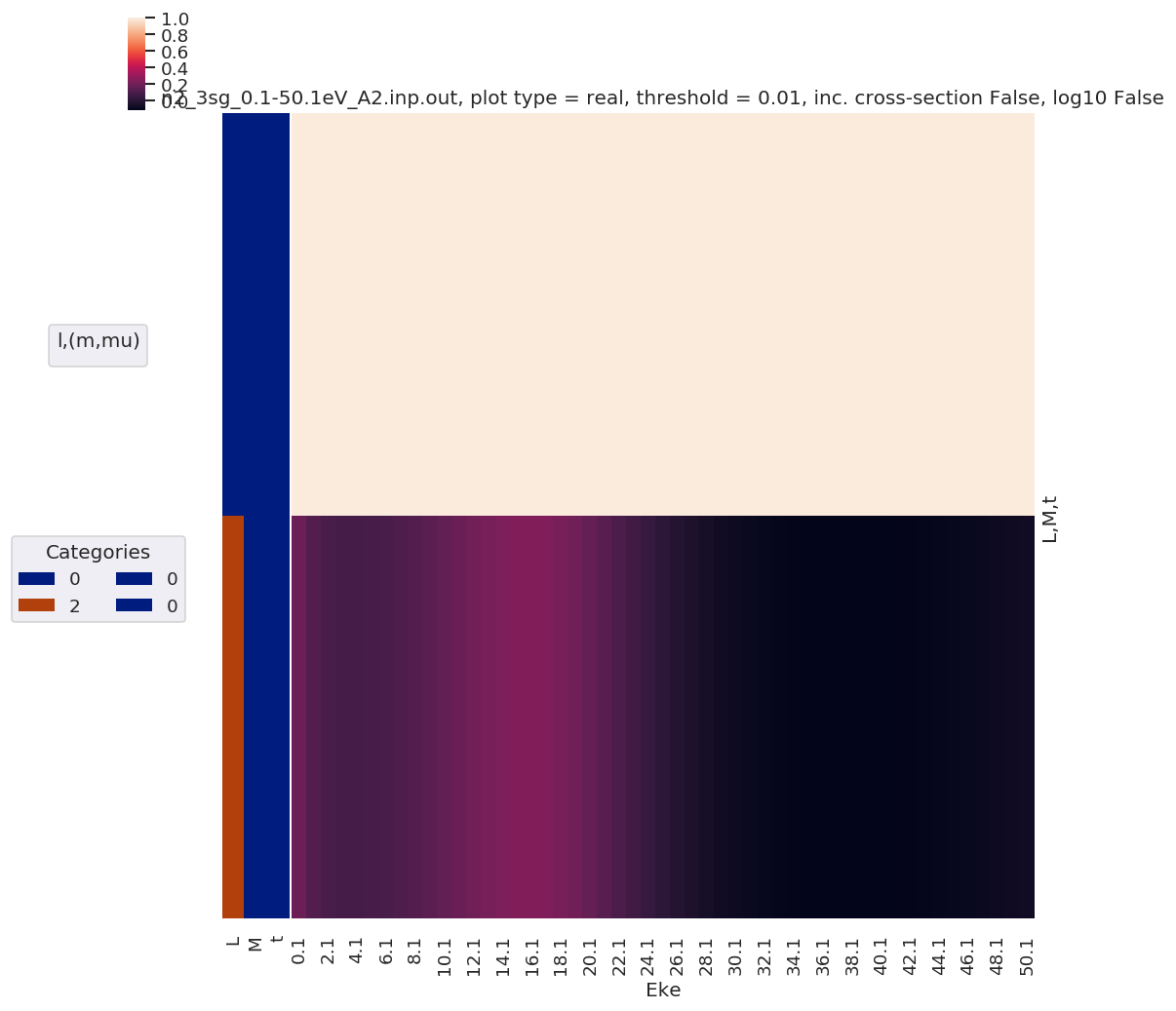:

mTermST.XS.real.squeeze().plot.line(x='Eke');:

ep.util.matEleSelector(mTermST, thres = 0.1, dims='Eke').real.squeeze().plot.line(x='Eke');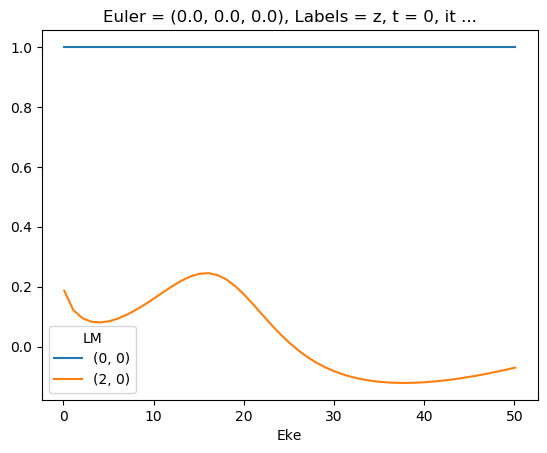First attempt… with sym summation

:

# ep.util.matEleSelector(mTermST, thres = 0.1, dims='Eke').real.squeeze().plot.line(x='Eke');
ep.util.matEleSelector(mTermST, thres = 0.1, dims='Eke').imag.squeeze().plot.line(x='Eke');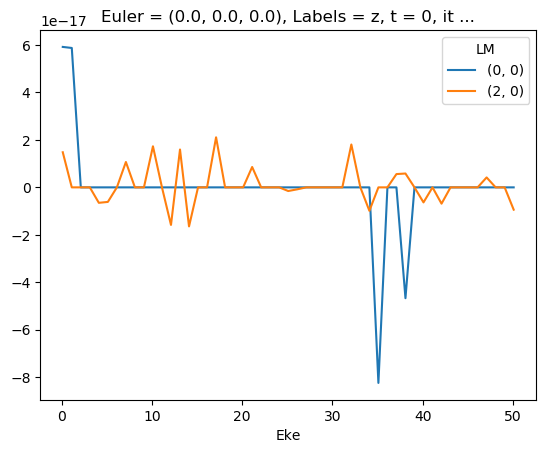### Test mult and renorm - seems like E-dependent XS issue here…¶

[ ]:



:

mTermTest = mTermST.copy()
# mTermTest.values = mTermTest*mTermTest.SF
# mTermTest.values = mTermTest/mTermTest.SF.pipe(np.abs)
mTermTest.values = mTermTest/mTermTest.SF
ep.util.matEleSelector(mTermTest, thres = 0.1, dims='Eke').real.squeeze().plot.line(x='Eke');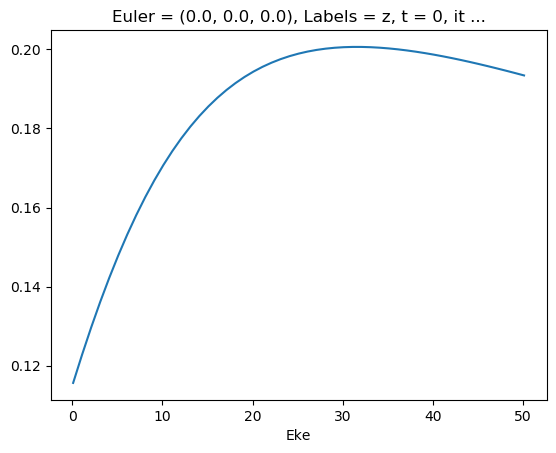:

ep.util.matEleSelector(mTermST['XS'], thres = 0.1, dims='Eke').real.squeeze().plot.line(x='Eke');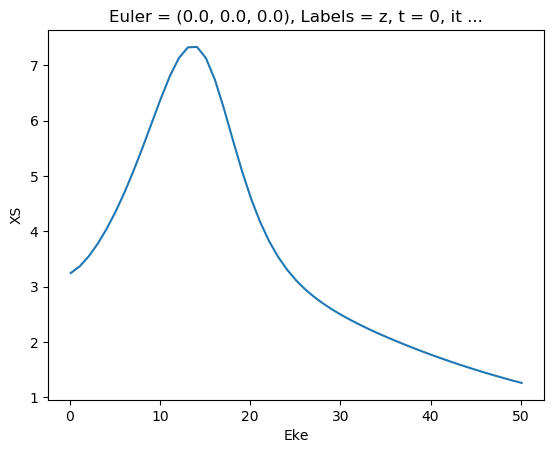:

ep.util.matEleSelector(mTermST['SF'], thres = 0.1, dims='Eke').real.squeeze().plot.line(x='Eke');
ep.util.matEleSelector(mTermST['SF'], thres = 0.1, dims='Eke').imag.squeeze().plot.line(x='Eke');
ep.util.matEleSelector(mTermST['SF'], thres = 0.1, dims='Eke').pipe(np.abs).squeeze().plot.line(x='Eke');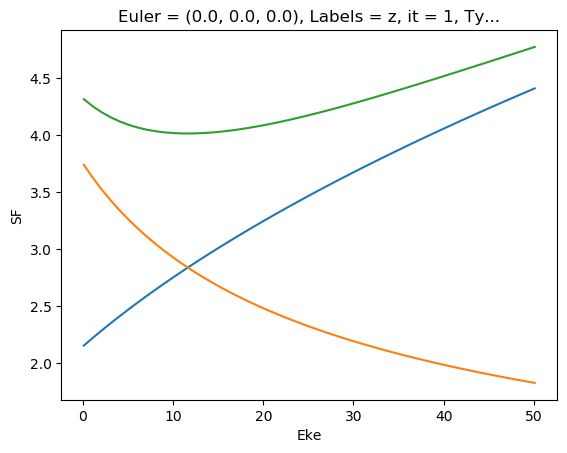:

mTermST.values = mTermST*mTermST.XS
ep.util.matEleSelector(mTermST, thres = 0.1, dims='Eke').real.squeeze().plot.line(x='Eke');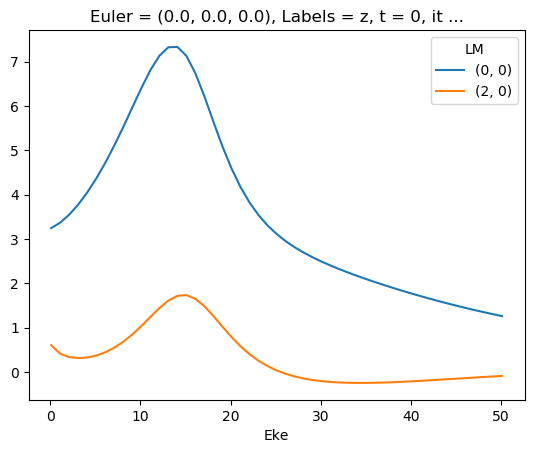:

matE.values = matE * matE.SF


## Test compared to experimental N2 AF results…¶

In this case MF PADs look good, so also expect good agreement with AF…

:

# Adapted from ePSproc_AFBLM_testing_010519_300719.m
# Load ADMs for N2
from scipy.io import loadmat
ADMdataFile = os.path.join(modPath, 'data', 'alignment', 'N2_ADM_VM_290816.mat')
ADMs = loadmat(ADMdataFile)

:

# Set tOffset for calcs, 3.76ps!!!
# This is because this is 2-pulse case, and will set t=0 to 2nd pulse (and matches defn. in N2 experimental paper)
tOffset = -3.76
ADMs['time'] = ADMs['time'] + tOffset

:

# Plot
import matplotlib.pyplot as plt
plt.plot(ADMs['time'].T, np.real(ADMs['ADM'].T))
plt.legend(ADMs['ADMlist'])
plt.xlabel('t/ps')
plt.ylabel('ADM')
plt.show()

:

[<matplotlib.lines.Line2D at 0x2c40580a0b8>,
<matplotlib.lines.Line2D at 0x2c4007392e8>,
<matplotlib.lines.Line2D at 0x2c400cc70f0>,
<matplotlib.lines.Line2D at 0x2c400cc7f28>]

:

<matplotlib.legend.Legend at 0x2c400960828>

:

Text(0.5, 0, 't/ps')

:

Text(0, 0.5, 'ADM')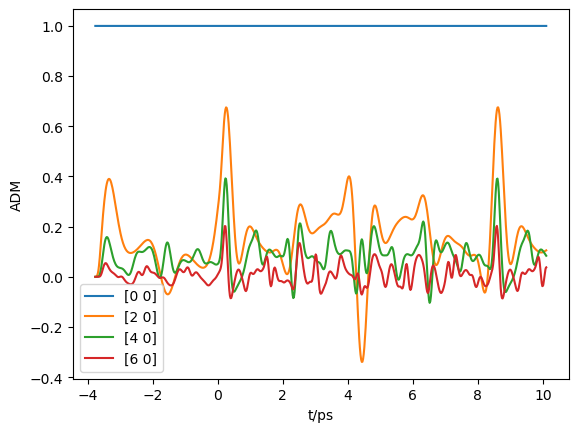:

# Selection & downsampling
trange=[1, 9.5]  # Set range in ps for calc
tStep=5  # Set tStep for downsampling

tMask = (ADMs['time']>trange) * (ADMs['time']<trange)
ind = np.nonzero(tMask)[0::tStep]
At = ADMs['time'][:,ind].squeeze()
ADMin = ADMs['ADM'][:,ind]

print(f"Selecting {ind.size} points")

plt.plot(At, np.real(ADMin.T))
plt.legend(ADMs['ADMlist'])
plt.xlabel('t/ps')
plt.ylabel('ADM')
plt.show()

# Set in Xarray
ADMX = ep.setADMs(ADMs = ADMs['ADM'][:,ind], t=At, KQSLabels = ADMs['ADMlist'], addS = True)
# ADMX

Selecting 87 points

:

[<matplotlib.lines.Line2D at 0x2c400b3b080>,
<matplotlib.lines.Line2D at 0x2c4724dea20>,
<matplotlib.lines.Line2D at 0x2c400b4f2e8>,
<matplotlib.lines.Line2D at 0x2c400b4f208>]

:

<matplotlib.legend.Legend at 0x2c4024c6908>

:

Text(0.5, 0, 't/ps')

:

Text(0, 0.5, 'ADM')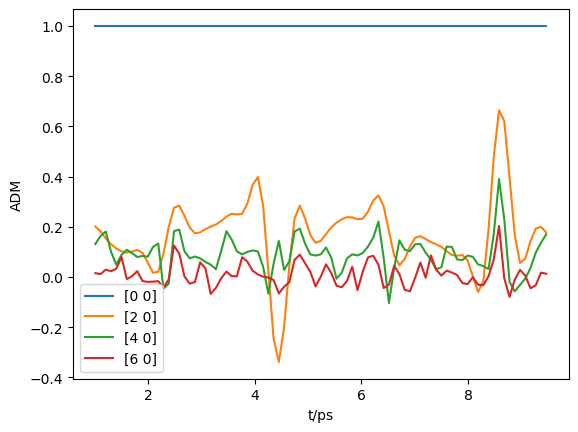:

# Run with sym summation...

phaseConvention = 'E'  # Set phase conventions used in the numerics - for ePolyScat matrix elements, set to 'E', to match defns. above.

symSum = True  # Sum over symmetry groups, or keep separate?
SFflag = False  # Include scaling factor to Mb in calculation?
BLMRenorm = False
thres = 1e-4
RX = ep.setPolGeoms()  # Set default pol geoms (z,x,y), or will be set by mfblmXprod() defaults - FOR AF case this is only used to set 'z' geom for unity wigner D's - should rationalise this!

start = time.time()
mTermST, mTermS, mTermTest = ep.geomFunc.afblmXprod(dataSet, QNs = None, AKQS=ADMX, RX=RX, thres = thres, selDims = {'it':1, 'Type':'L'}, thresDims='Eke',
symSum=symSum, SFflag=SFflag, BLMRenorm = BLMRenorm,
phaseConvention=phaseConvention)
end = time.time()
print('Elapsed time = {0} seconds, for {1} energy points, {2} polarizations, threshold={3}.'.format((end-start), mTermST.Eke.size, RX.size, thres))

# Elapsed time = 3.3885273933410645 seconds, for 51 energy points, 3 polarizations, threshold=0.01.
# Elapsed time = 5.059587478637695 seconds, for 51 energy points, 3 polarizations, threshold=0.0001.

Elapsed time = 3.8453733921051025 seconds, for 51 energy points, 3 polarizations, threshold=0.0001.

:

# Full results (before summation)
mTermST.attrs['dataType'] = 'matE'  # Set matE here to allow for correct plotting of sym dims.

plotDimsRed = ['Labels','L','M']  # Set plotDims to fix dim ordering in plot
if not symSum:
plotDimsRed.extend(['Cont','Targ','Total'])

# daPlot, daPlotpd, legendList, gFig = ep.lmPlot(mTermST, plotDims=plotDimsRed, xDim='Eke', sumDims=None, pType = 'r', thres = 0.01, fillna = True, SFflag=False)

# daPlot, daPlotpd, legendList, gFig = ep.lmPlot(mTermST, xDim='Eke', sumDims=None, pType = 'r', thres = 0.01, fillna = True)  # If plotDims is not passed use default ordering.

:

# daPlot, daPlotpd, legendList, gFig = ep.lmPlot(mTermST.XS, plotDims=plotDimsRed, xDim='Eke', sumDims=None, pType = 'r', thres = 0.01, fillna = True, SFflag=False)

:

mTermST.XS.real.plot()
mTermST.where(mTermST.L > 0).real.plot(col='LM')

:

<matplotlib.collections.QuadMesh at 0x2c400c7a0b8>

:

<xarray.plot.facetgrid.FacetGrid at 0x2c4058c19e8>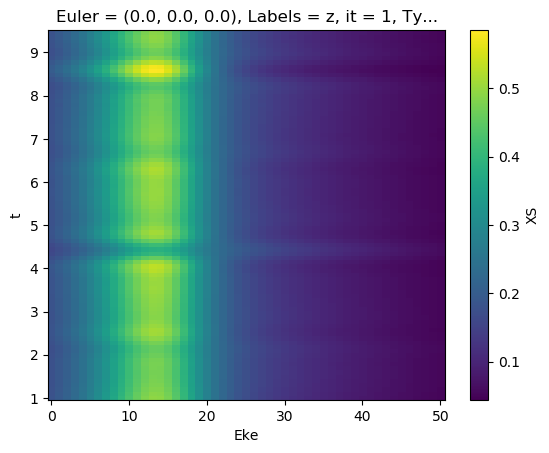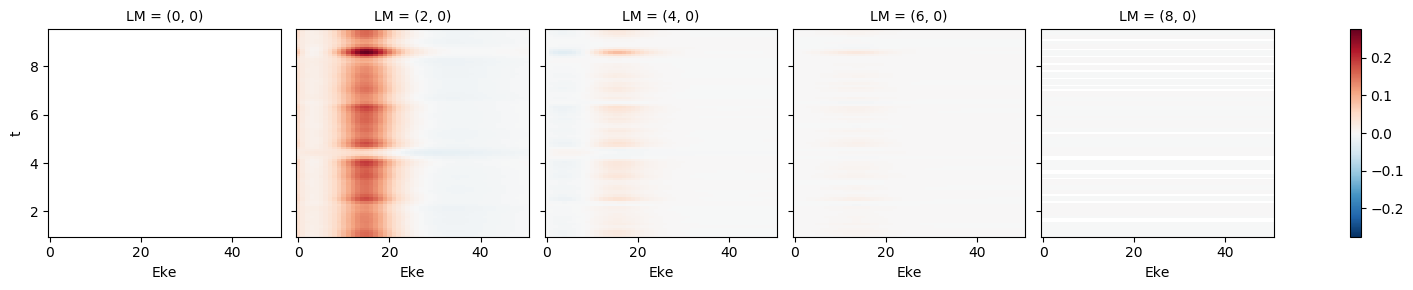:

# Plot single energy XS
E = 8.1
mTermST.XS.sel({'Eke':E}).real.squeeze().plot.line(x='t');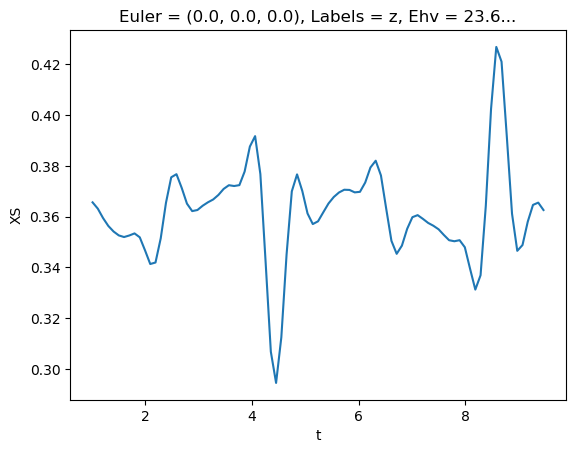:

# LM with renorm
mTermST.sel({'Eke':E}).where(mTermST['L']>0).real.squeeze().plot.line(x='t');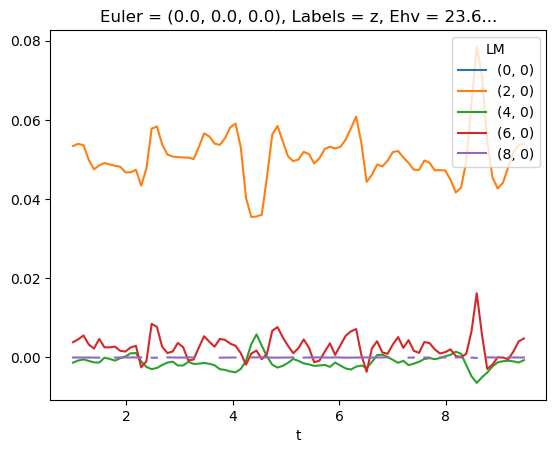:

# LM * XS
# (mTermST * mTermST.XS).sel({'Eke':E}).where(mTermST['L']>0).real.squeeze().plot.line(x='t');


## Experimental results¶

(Fig 2 from Marceau, Claude, Varun Makhija, Dominique Platzer, A. Yu. Naumov, P. B. Corkum, Albert Stolow, D. M. Villeneuve, and Paul Hockett. “Molecular Frame Reconstruction Using Time-Domain Photoionization Interferometry.” Physical Review Letters 119, no. 8 (August 2017): 083401. https://doi.org/10.1103/PhysRevLett.119.083401. Full data at https://doi.org/10.6084/m9.figshare.4480349.v10)

Currently:

• Relative signs/phases of betas look good at 4.1eV… but not at 7.1 or 8.1eV! (Experimental MFPAD comparison energy) - suggests still phase and/or renorm issue somewhere?
• Values still off, same issue probably.
• Might be phase issue with sum over rotation matrix elements, which are conj() in MF case, but not in original derivation here…? This could give 3j sign-flip, hence change calculated alignment response.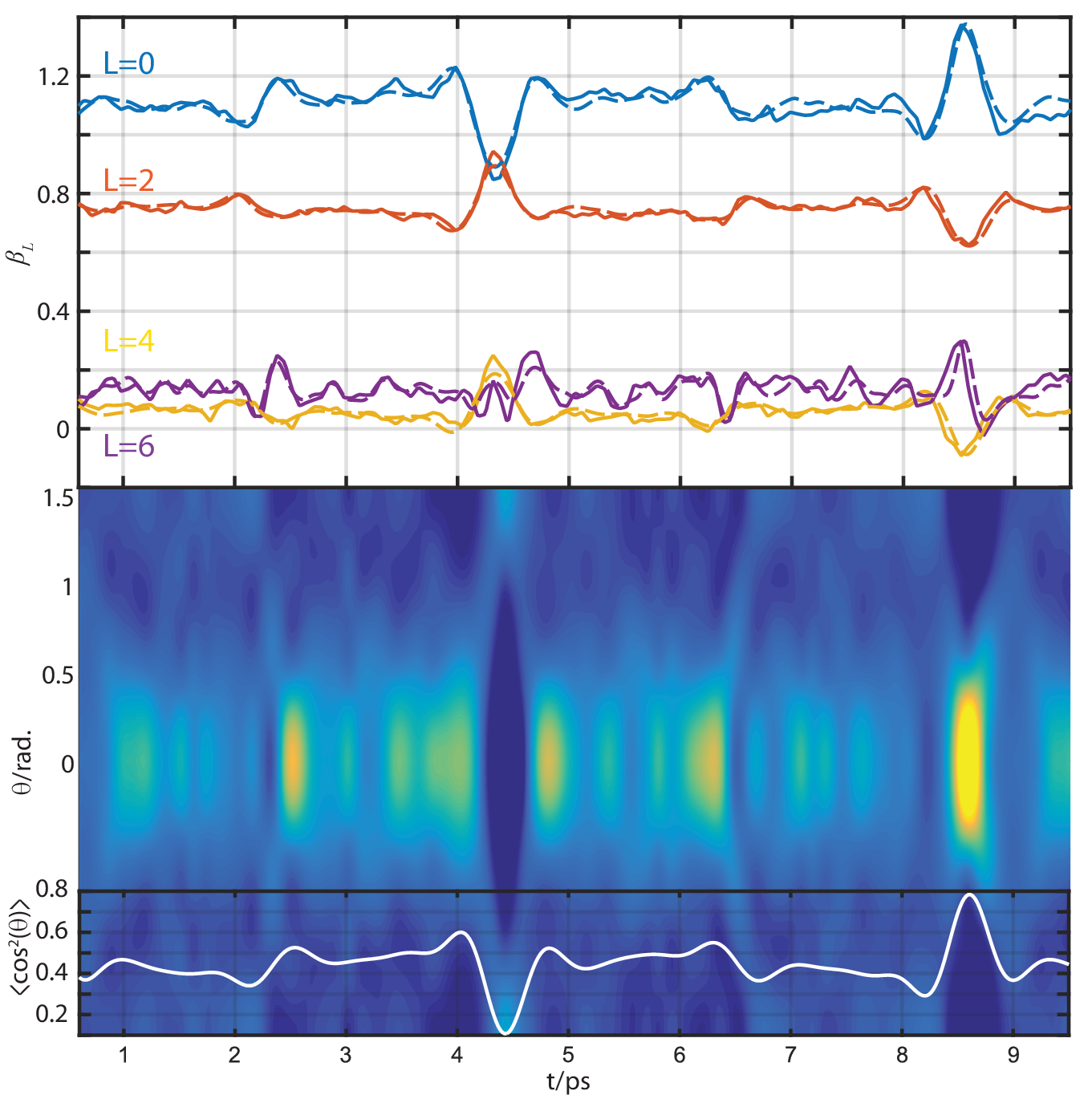# SCRATCH¶

:

# Phase switch example
if phaseCons['mfblmCons']['BLMmPhase']:
QNsBLMtable[:,3] *= -1
QNsBLMtable[:,5] *= -1

  File "<ipython-input-62-6783c300192b>", line 2
if phaseCons['mfblmCons']['BLMmPhase']:
^
IndentationError: unexpected indent


[ ]:


def deltaKQS(QNs = None):
phaseCons = setPhaseConventions(phaseConvention = phaseConvention)

# If no QNs are passed, set for all possible terms
if QNs is None:
QNs = []

# Set photon terms
l = 1
lp = 1

# Loop to set all other QNs
for mu in np.arange(-l, l+1):
for mup in np.arange(-lp, lp+1):
#for R in np.arange(-(l+lp)-1, l+lp+2):
#    for P in np.arange(0, l+lp+1):
for P in np.arange(0, l+lp+1):
# for Rp in np.arange(-P, P+1):  # Allow all Rp
# Rp = -(mu+mup)   # Fix Rp terms - not valid here, depends on other phase cons!
# for R in np.arange(-P, P+1):
#     # QNs.append([l, lp, P, mu, -mup, R, Rp])
#     if phaseCons['lambdaCons']['negRp']:
#         Rp *= -1
#     if phaseCons['lambdaCons']['negMup']:
#         QNs.append([l, lp, P, mu, -mup, Rp, R])   # 31/03/20: FIXED bug, (R,Rp) previously misordered!!!
#     else:
#         QNs.append([l, lp, P, mu, mup, Rp, R])

# Rearranged for specified Rp case
for R in np.arange(-P, P+1):
# if phaseCons['lambdaCons']['negMup']:
#     mup = -mup

if phaseCons['lambdaCons']['negRp']:
# Rp = mu+mup
Rp = mup - mu
else:
Rp = -(mu+mup)

# Switch mup sign for 3j?  To match old numerics, this is *after* Rp assignment (sigh).
if phaseCons['lambdaCons']['negMup']:
mup = -mup

QNs.append([l, lp, P, mu, mup, Rp, R])

QNs = np.array(QNs)